Скачать презентацию Chapter 2 One-Dimensional Kinematics Kinematics It

d3a25a6f8043b1e768d6e03fa3840b6f.ppt

• Количество слайдов: 85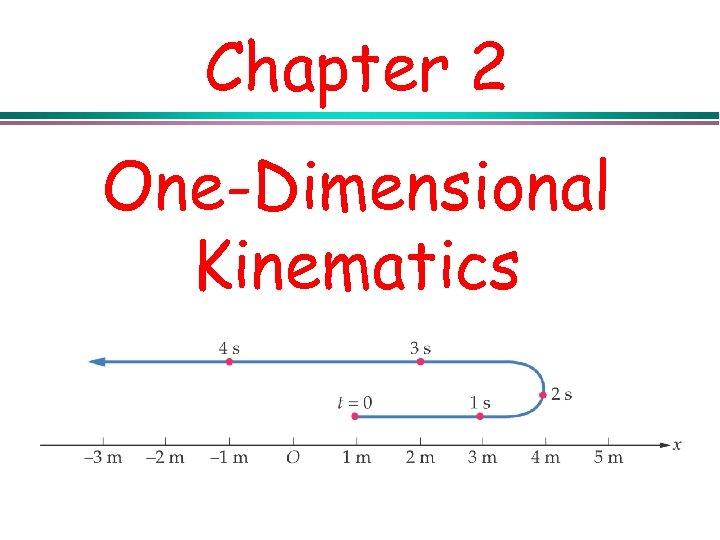Chapter 2 One-Dimensional Kinematics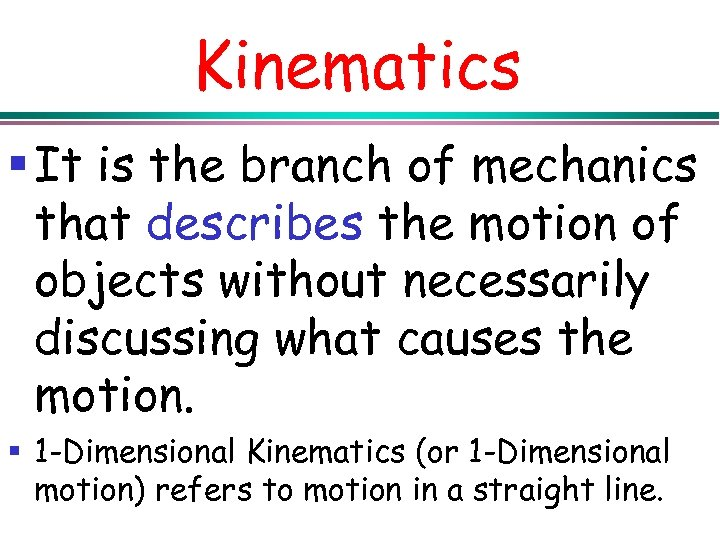Kinematics § It is the branch of mechanics that describes the motion of objects without necessarily discussing what causes the motion. § 1 -Dimensional Kinematics (or 1 -Dimensional motion) refers to motion in a straight line.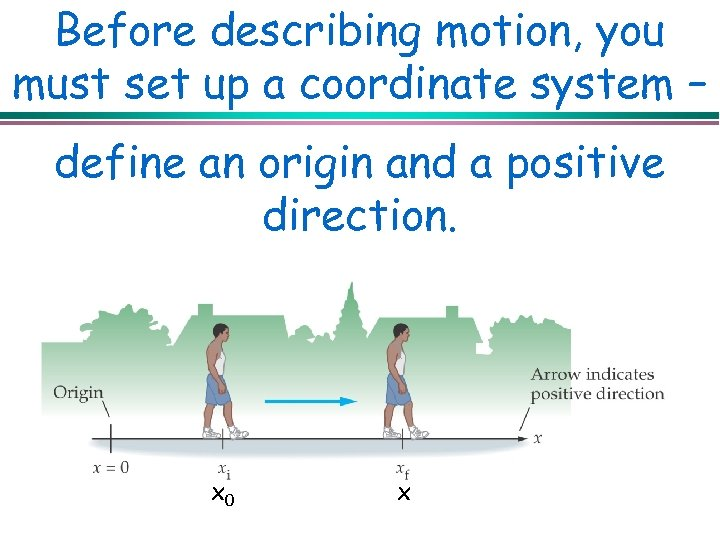Before describing motion, you must set up a coordinate system – define an origin and a positive direction. x 0 x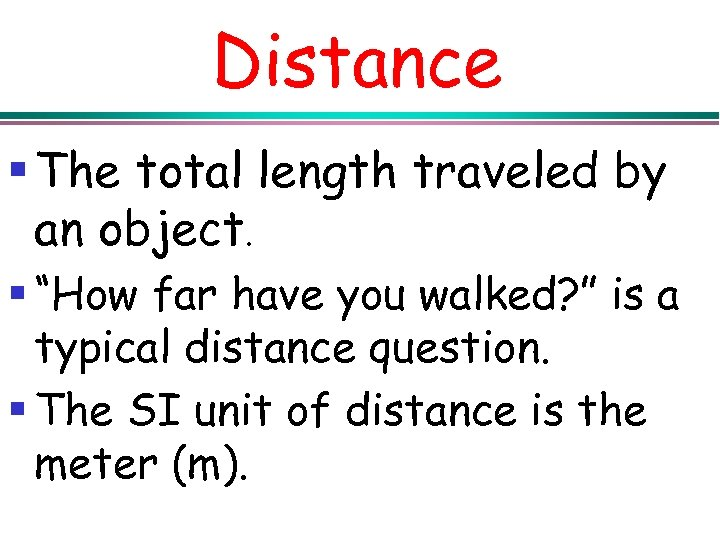Distance § The total length traveled by an object. § “How far have you walked? ” is a typical distance question. § The SI unit of distance is the meter (m).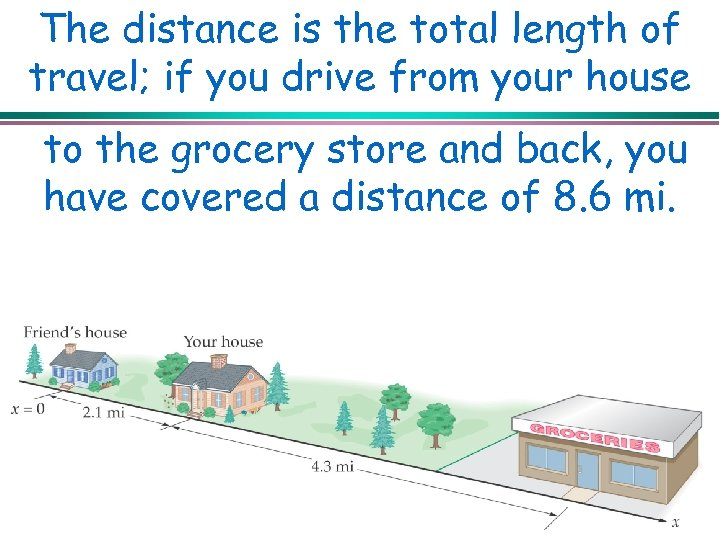The distance is the total length of travel; if you drive from your house to the grocery store and back, you have covered a distance of 8. 6 mi.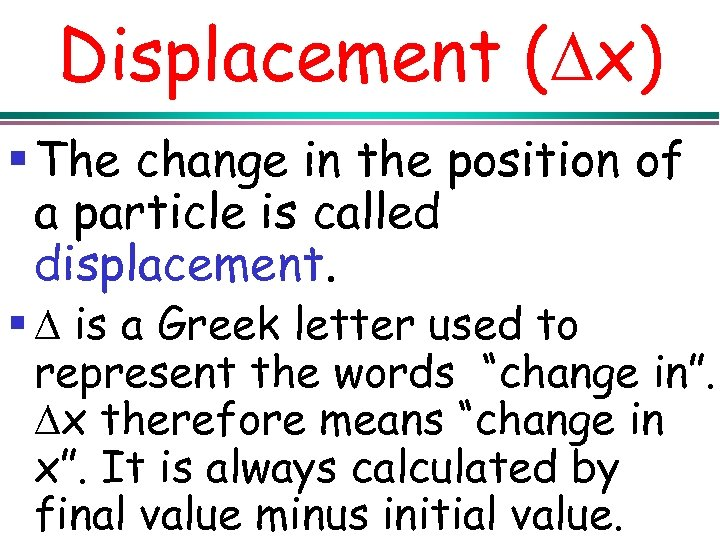Displacement ( x) § The change in the position of a particle is called displacement. § is a Greek letter used to represent the words “change in”. x therefore means “change in x”. It is always calculated by final value minus initial value.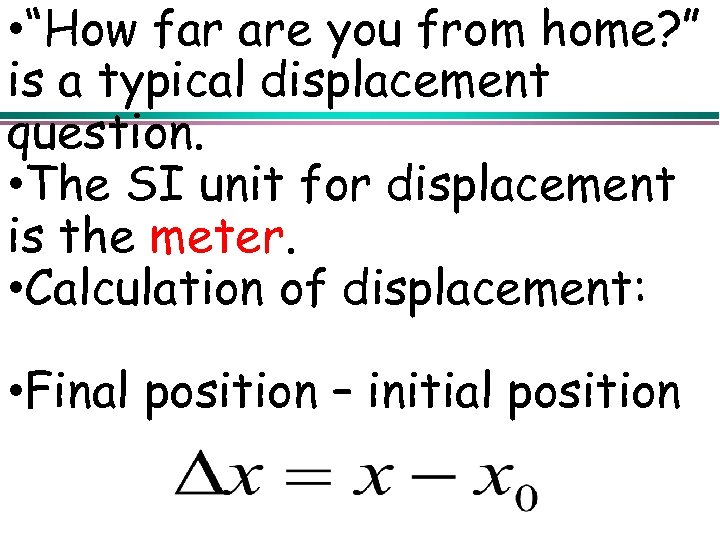• “How far are you from home? ” is a typical displacement question. • The SI unit for displacement is the meter. • Calculation of displacement: • Final position – initial position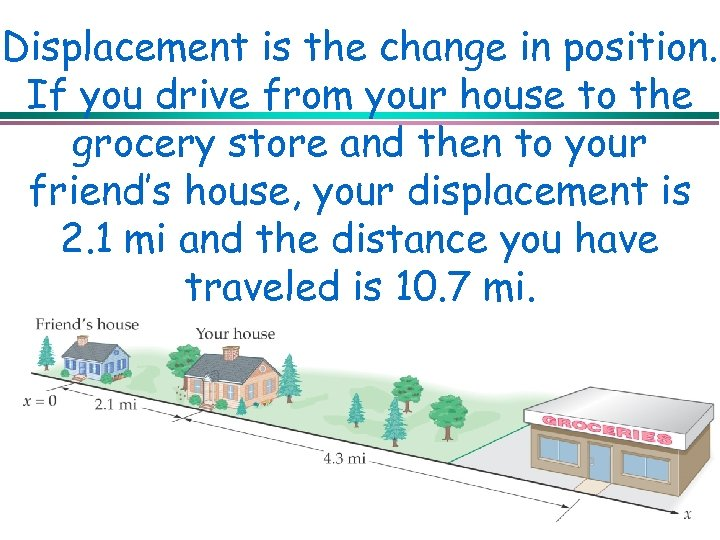Displacement is the change in position. If you drive from your house to the grocery store and then to your friend’s house, your displacement is 2. 1 mi and the distance you have traveled is 10. 7 mi.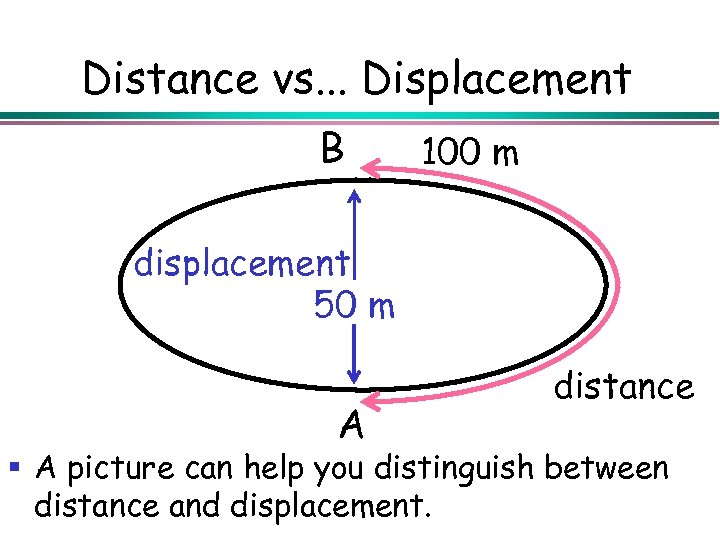Distance vs. . . Displacement B 100 m displacement 50 m A distance § A picture can help you distinguish between distance and displacement.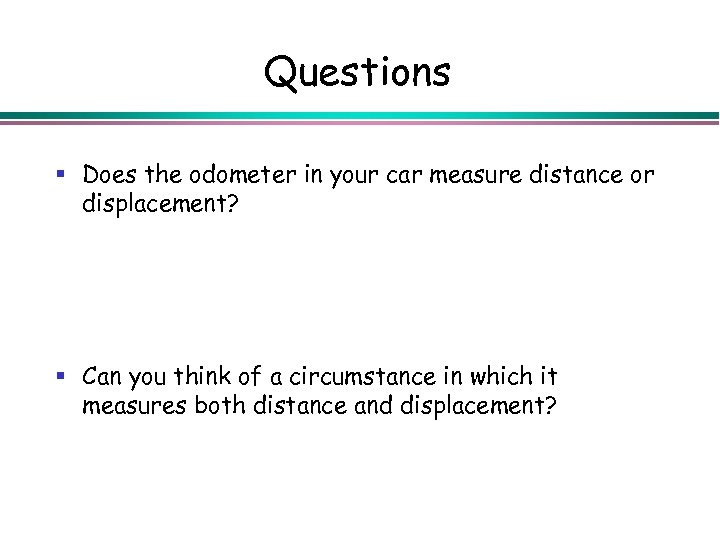Questions § Does the odometer in your car measure distance or displacement? § Can you think of a circumstance in which it measures both distance and displacement?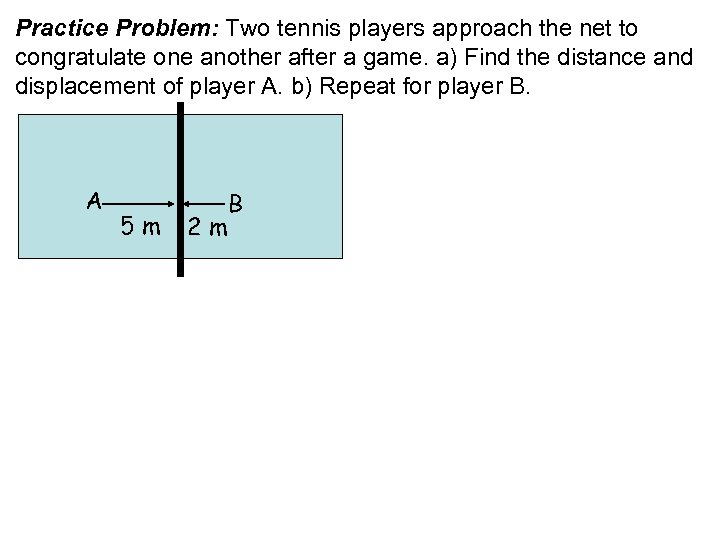Practice Problem: Two tennis players approach the net to congratulate one another after a game. a) Find the distance and displacement of player A. b) Repeat for player B. A 5 m 2 m B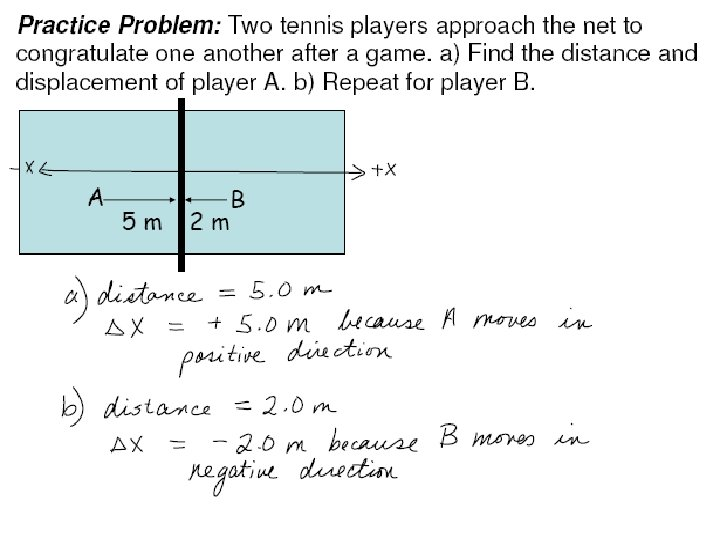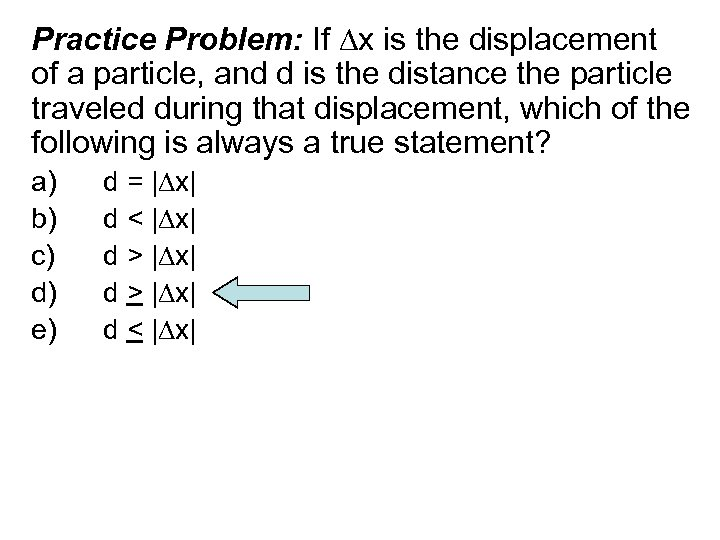Practice Problem: If x is the displacement of a particle, and d is the distance the particle traveled during that displacement, which of the following is always a true statement? a) b) c) d) e) d = | x| d < | x| d > | x| d < | x|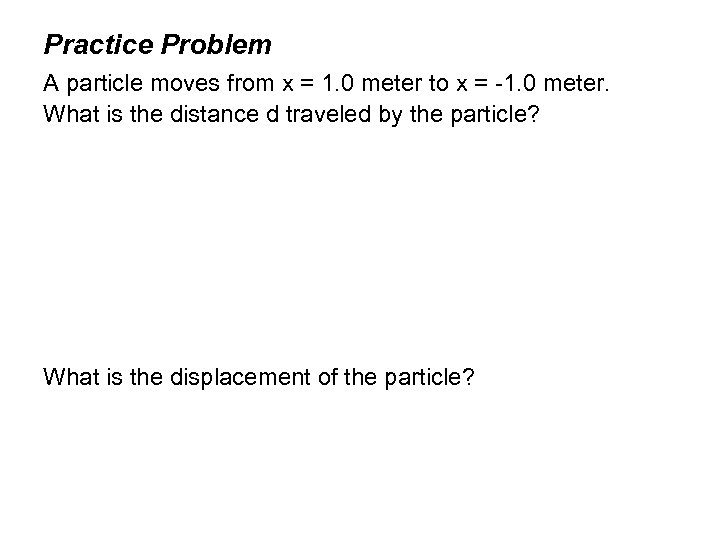Practice Problem A particle moves from x = 1. 0 meter to x = -1. 0 meter. What is the distance d traveled by the particle? What is the displacement of the particle?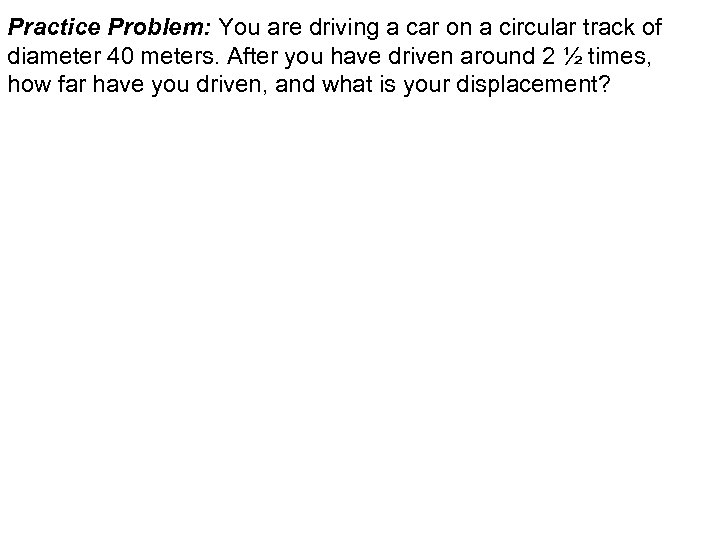Practice Problem: You are driving a car on a circular track of diameter 40 meters. After you have driven around 2 ½ times, how far have you driven, and what is your displacement?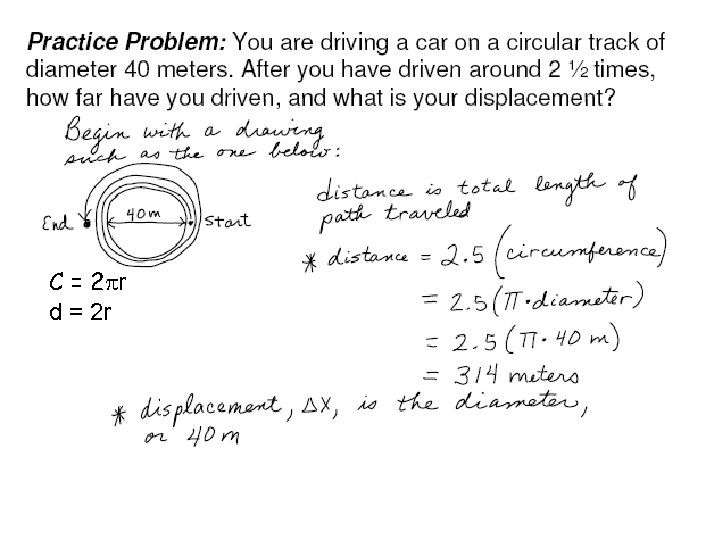C = 2 pr d = 2 r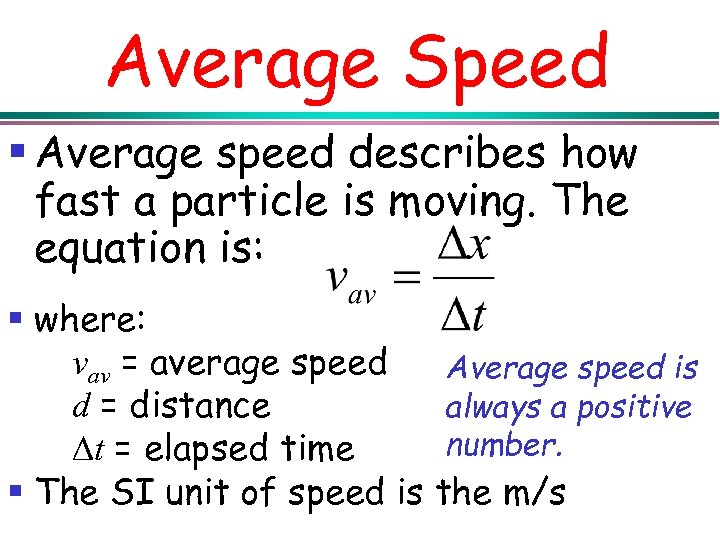Average Speed § Average speed describes how fast a particle is moving. The equation is: § where: vav = average speed Average speed is always a positive d = distance number. t = elapsed time § The SI unit of speed is the m/s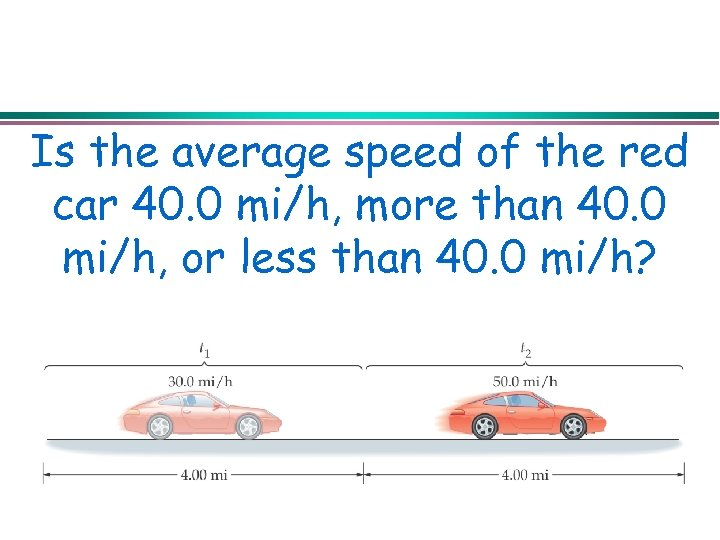Is the average speed of the red car 40. 0 mi/h, more than 40. 0 mi/h, or less than 40. 0 mi/h?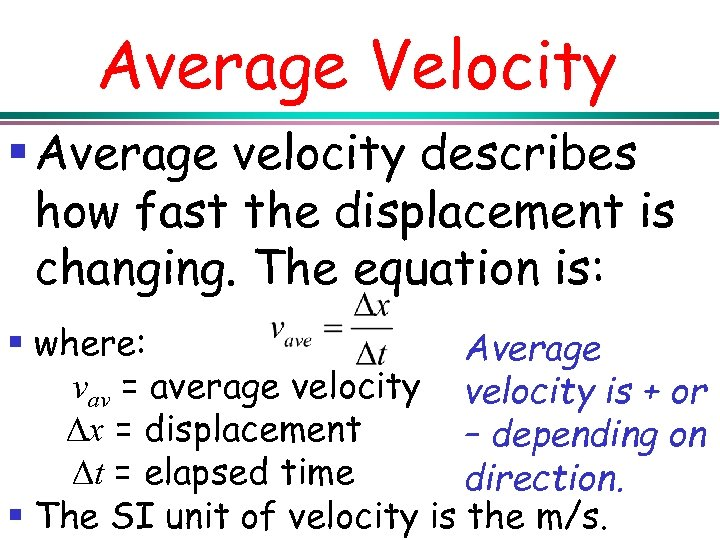Average Velocity § Average velocity describes how fast the displacement is changing. The equation is: § where: Average vav = average velocity is + or x = displacement – depending on t = elapsed time direction. § The SI unit of velocity is the m/s.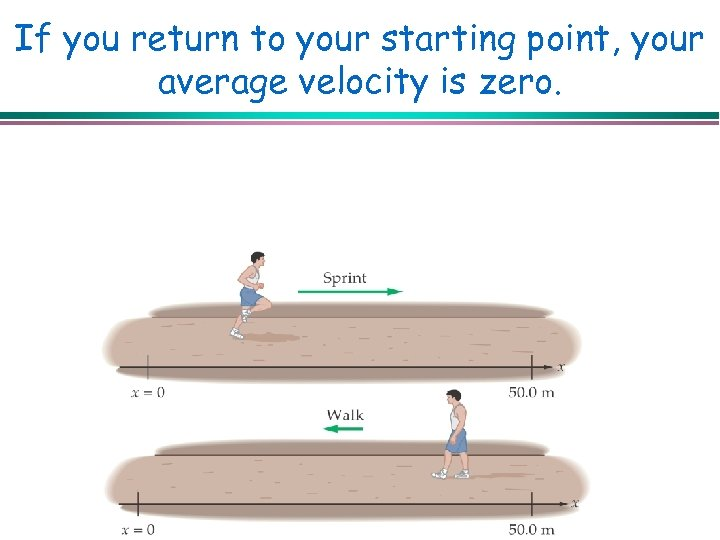If you return to your starting point, your average velocity is zero.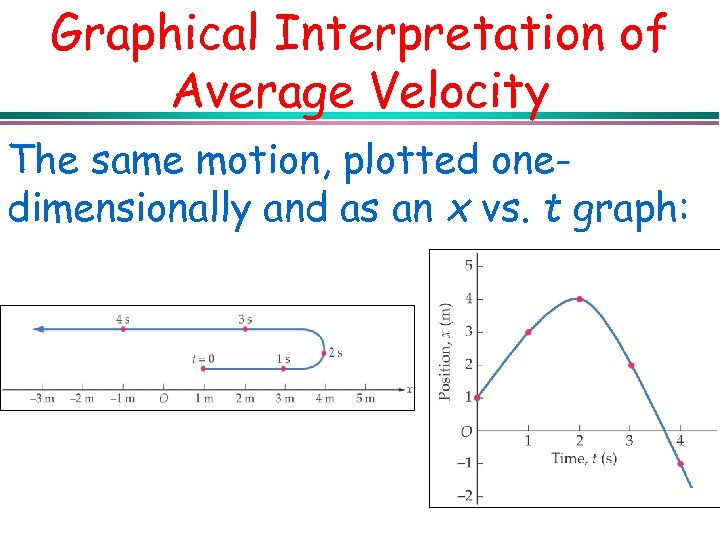Graphical Interpretation of Average Velocity The same motion, plotted onedimensionally and as an x vs. t graph: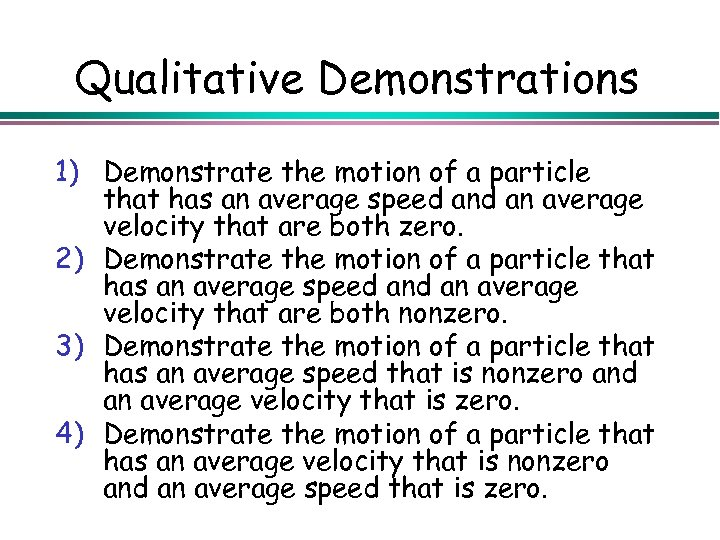Qualitative Demonstrations 1) Demonstrate the motion of a particle that has an average speed an average velocity that are both zero. 2) Demonstrate the motion of a particle that has an average speed an average velocity that are both nonzero. 3) Demonstrate the motion of a particle that has an average speed that is nonzero and an average velocity that is zero. 4) Demonstrate the motion of a particle that has an average velocity that is nonzero and an average speed that is zero.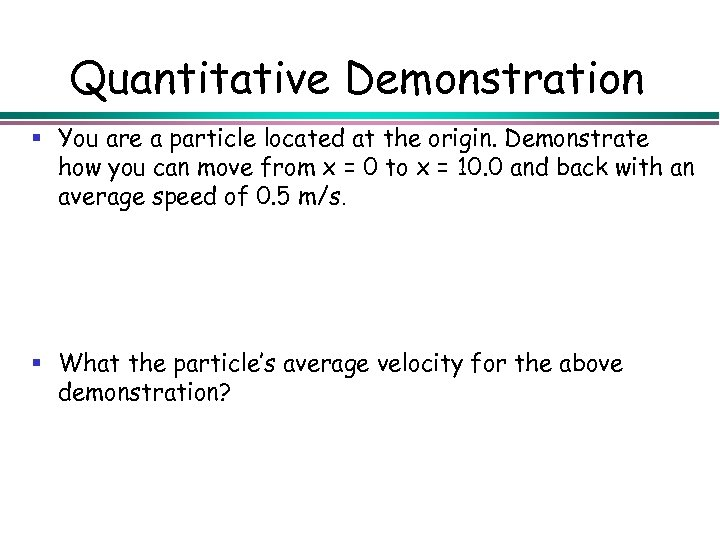Quantitative Demonstration § You are a particle located at the origin. Demonstrate how you can move from x = 0 to x = 10. 0 and back with an average speed of 0. 5 m/s. § What the particle’s average velocity for the above demonstration?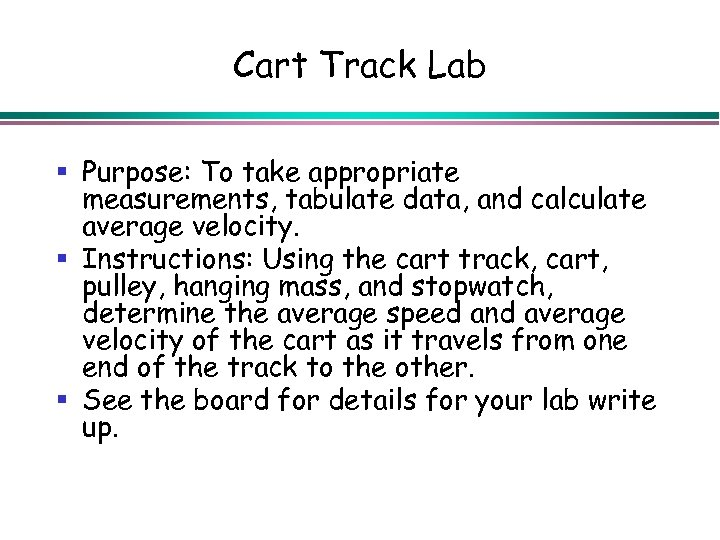Cart Track Lab § Purpose: To take appropriate measurements, tabulate data, and calculate average velocity. § Instructions: Using the cart track, cart, pulley, hanging mass, and stopwatch, determine the average speed and average velocity of the cart as it travels from one end of the track to the other. § See the board for details for your lab write up.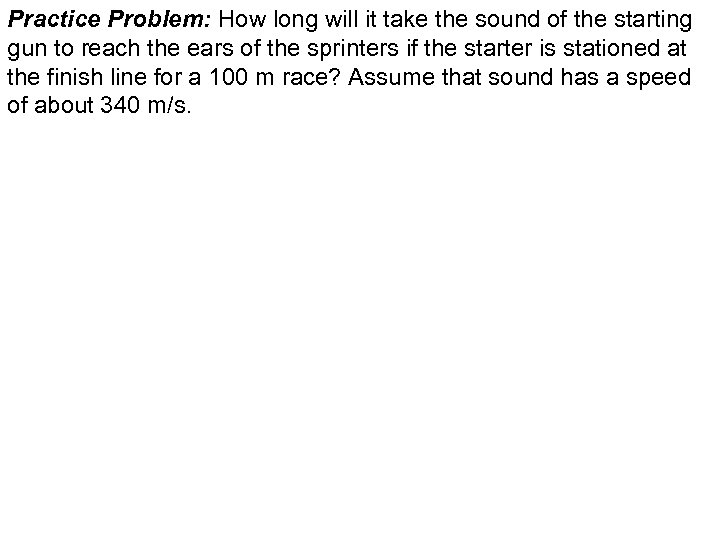Practice Problem: How long will it take the sound of the starting gun to reach the ears of the sprinters if the starter is stationed at the finish line for a 100 m race? Assume that sound has a speed of about 340 m/s.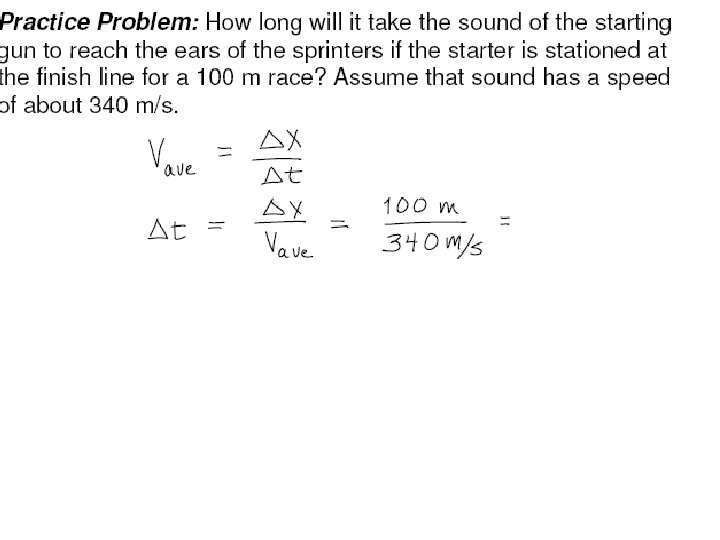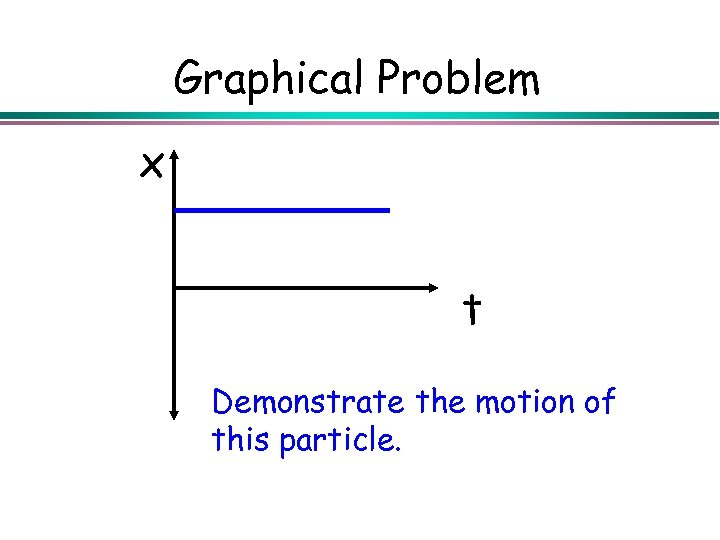Graphical Problem x t Demonstrate the motion of this particle.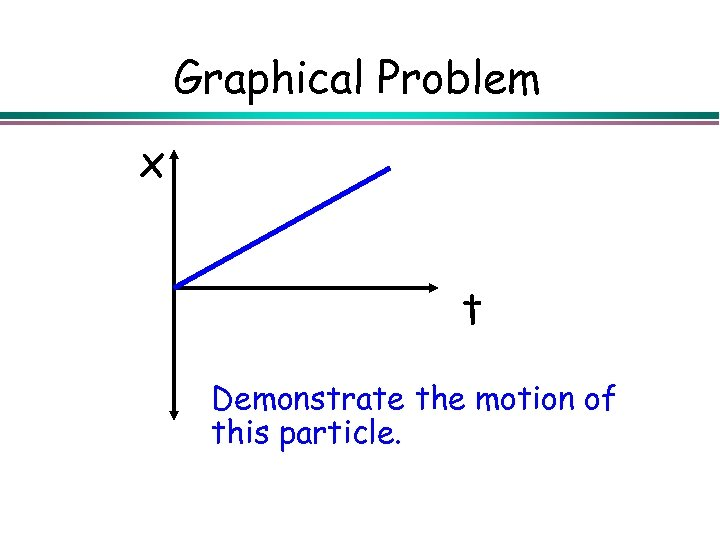Graphical Problem x t Demonstrate the motion of this particle.Graphical Problem x B A x t vav = x/ t t What physical feature of the graph gives the constant velocity from A to B?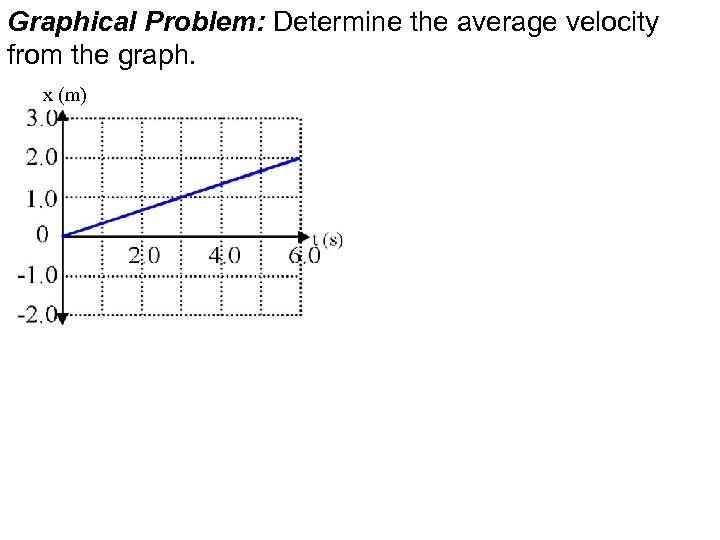Graphical Problem: Determine the average velocity from the graph. x (m)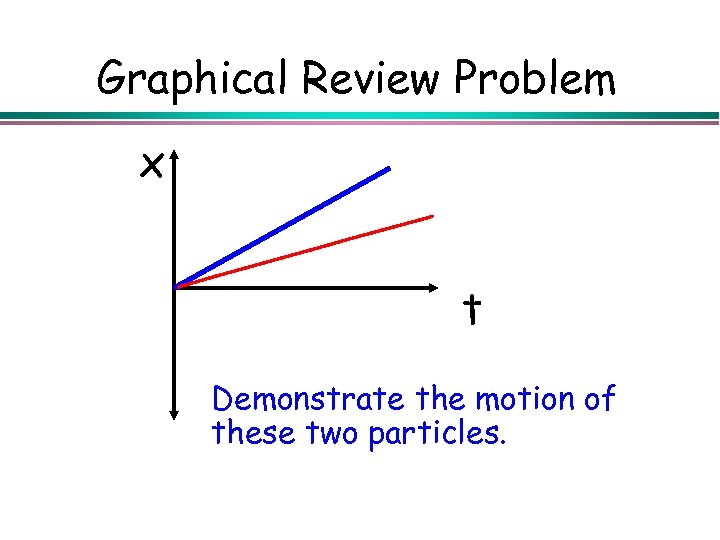Graphical Review Problem x t Demonstrate the motion of these two particles.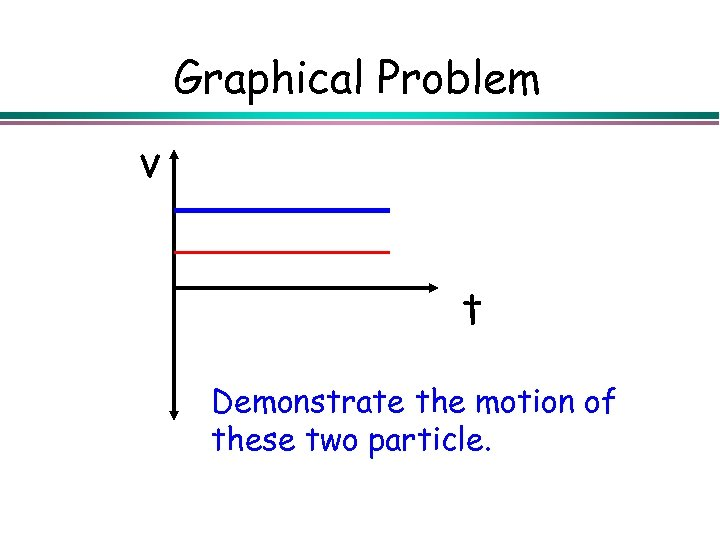Graphical Problem v t Demonstrate the motion of these two particle.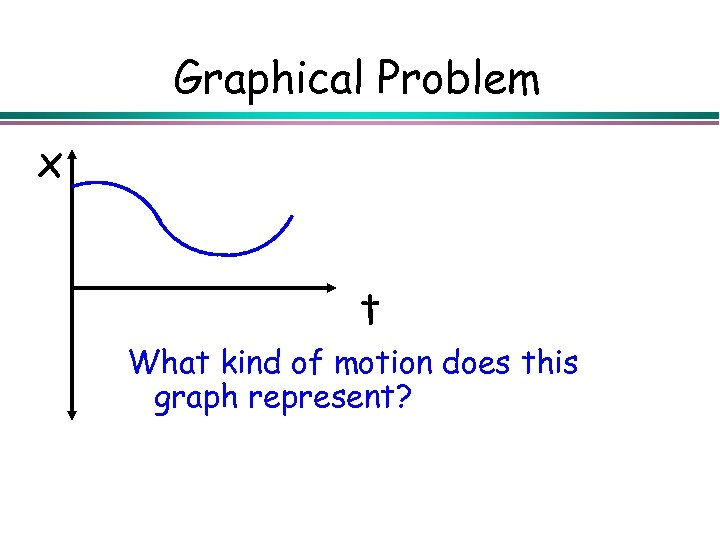Graphical Problem x t What kind of motion does this graph represent?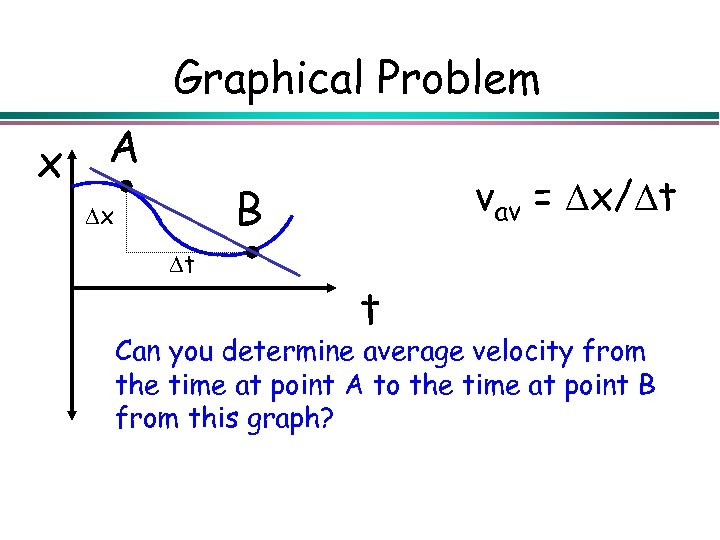Graphical Problem x A vav = x/ t B x t t Can you determine average velocity from the time at point A to the time at point B from this graph?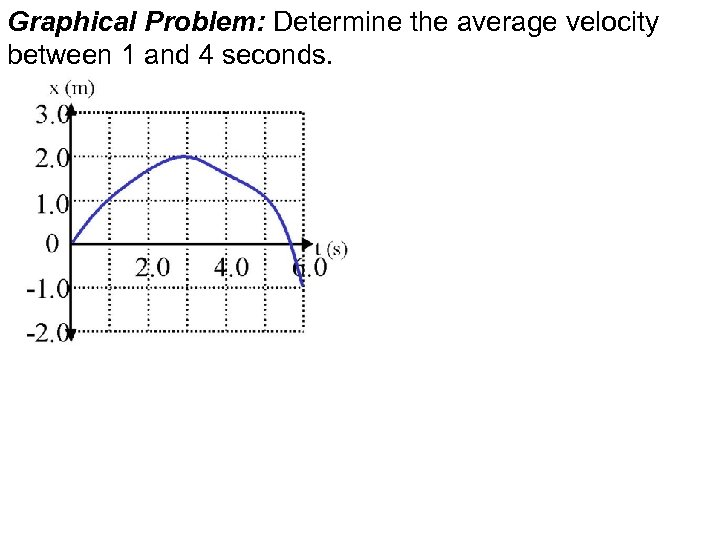Graphical Problem: Determine the average velocity between 1 and 4 seconds.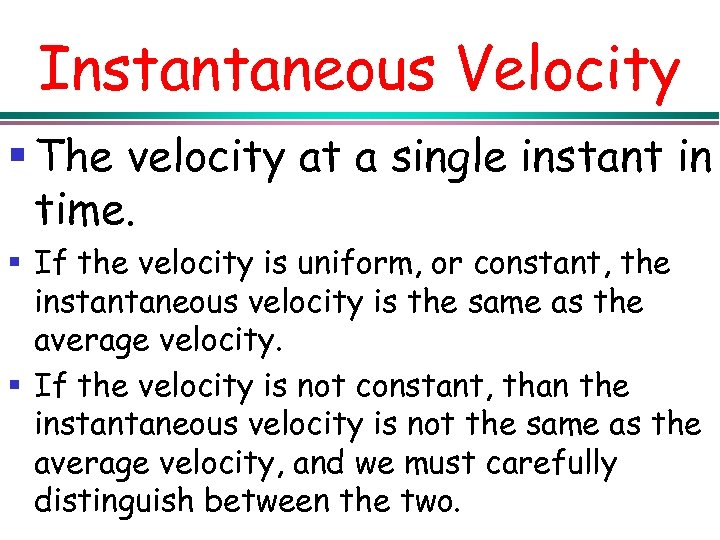Instantaneous Velocity § The velocity at a single instant in time. § If the velocity is uniform, or constant, the instantaneous velocity is the same as the average velocity. § If the velocity is not constant, than the instantaneous velocity is not the same as the average velocity, and we must carefully distinguish between the two.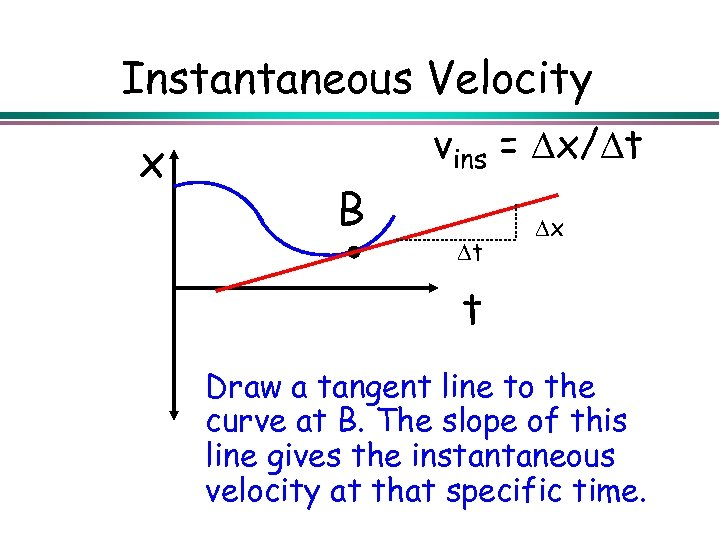Instantaneous Velocity x vins = x/ t B t x t Draw a tangent line to the curve at B. The slope of this line gives the instantaneous velocity at that specific time.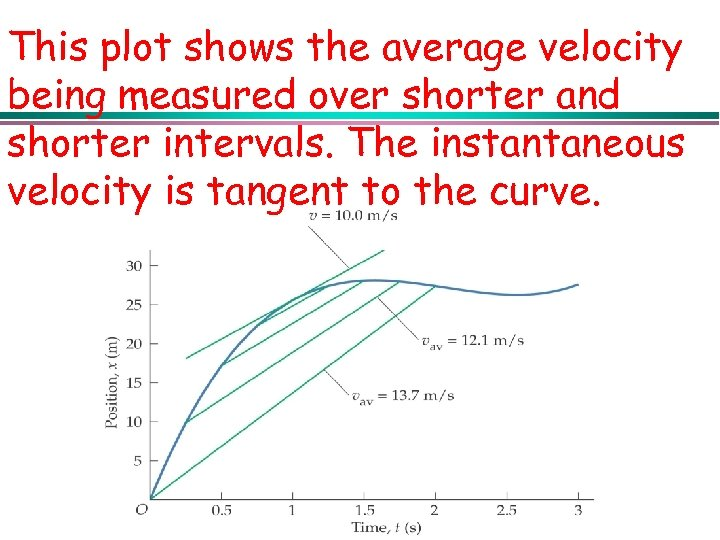This plot shows the average velocity being measured over shorter and shorter intervals. The instantaneous velocity is tangent to the curve.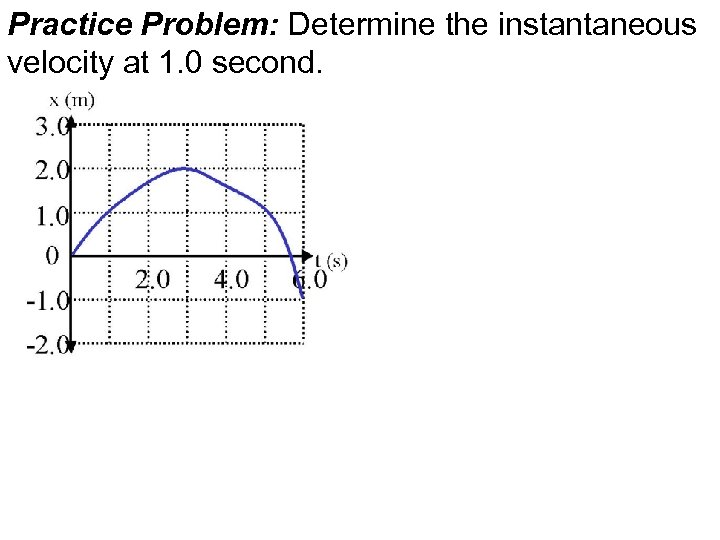Practice Problem: Determine the instantaneous velocity at 1. 0 second.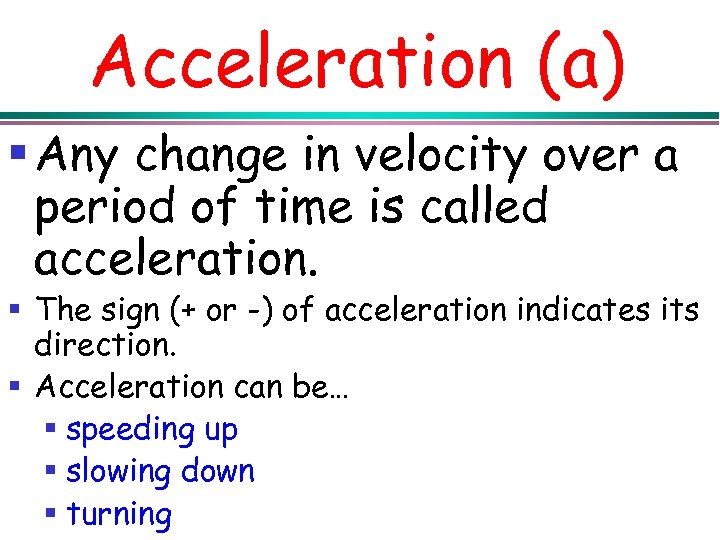Acceleration (a) § Any change in velocity over a period of time is called acceleration. § The sign (+ or -) of acceleration indicates its direction. § Acceleration can be… § speeding up § slowing down § turning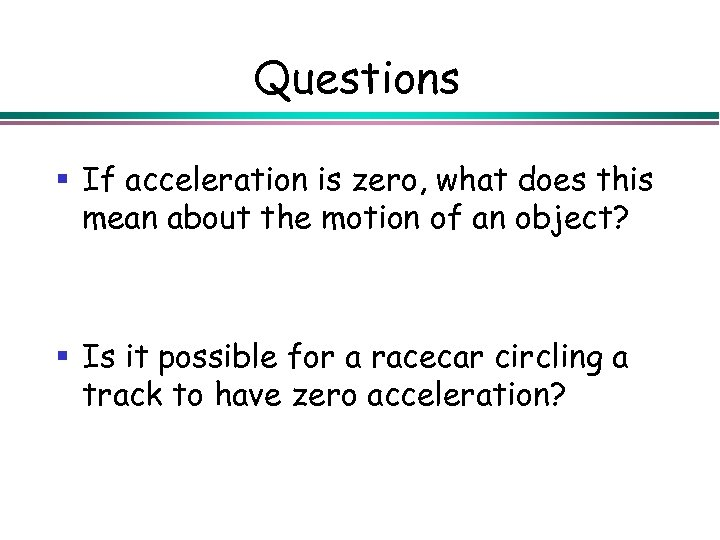Questions § If acceleration is zero, what does this mean about the motion of an object? § Is it possible for a racecar circling a track to have zero acceleration?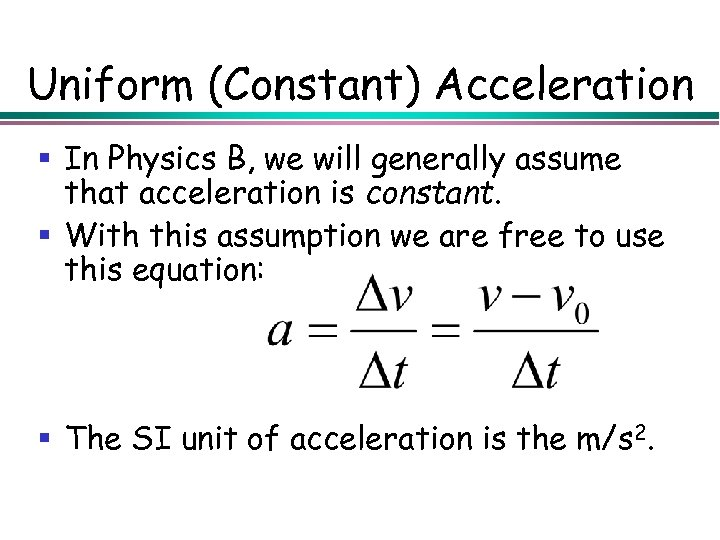Uniform (Constant) Acceleration § In Physics B, we will generally assume that acceleration is constant. § With this assumption we are free to use this equation: § The SI unit of acceleration is the m/s 2.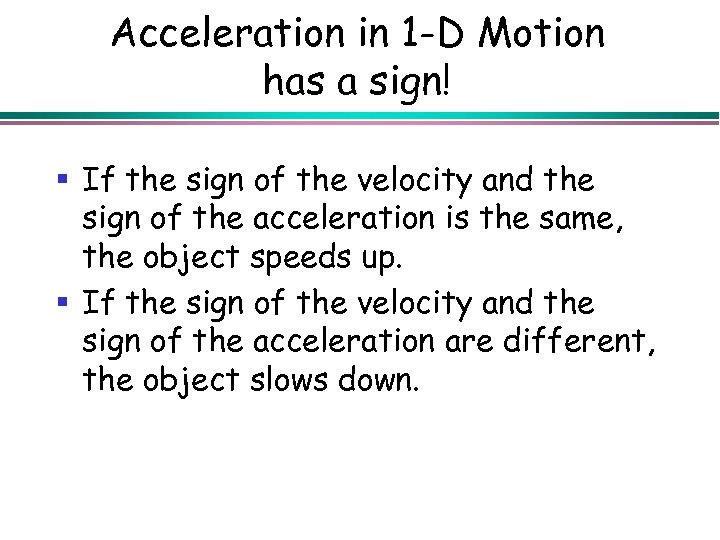Acceleration in 1 -D Motion has a sign! § If the sign of the velocity and the sign of the acceleration is the same, the object speeds up. § If the sign of the velocity and the sign of the acceleration are different, the object slows down.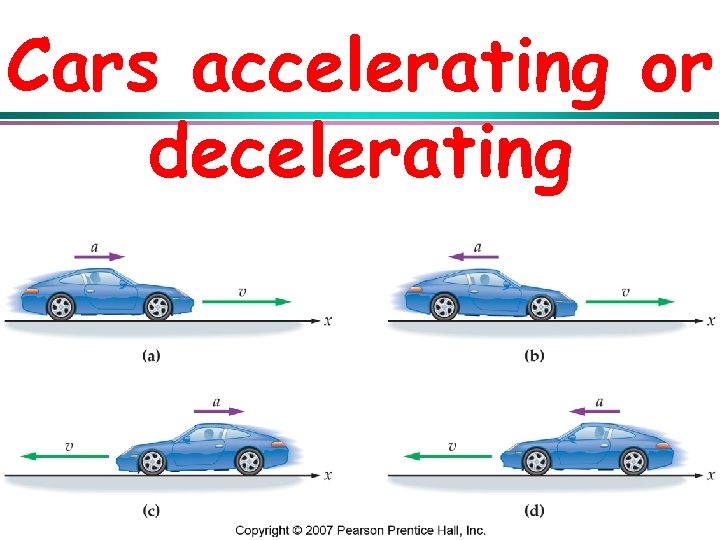Cars accelerating or decelerating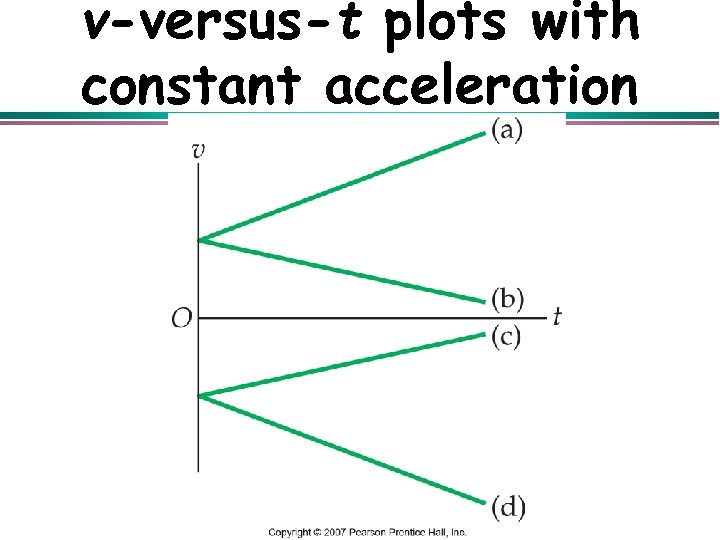v-versus-t plots with constant acceleration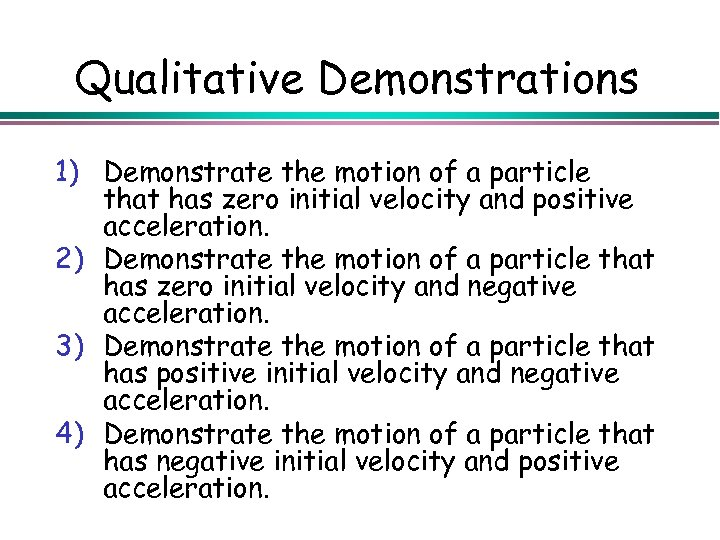Qualitative Demonstrations 1) Demonstrate the motion of a particle that has zero initial velocity and positive acceleration. 2) Demonstrate the motion of a particle that has zero initial velocity and negative acceleration. 3) Demonstrate the motion of a particle that has positive initial velocity and negative acceleration. 4) Demonstrate the motion of a particle that has negative initial velocity and positive acceleration.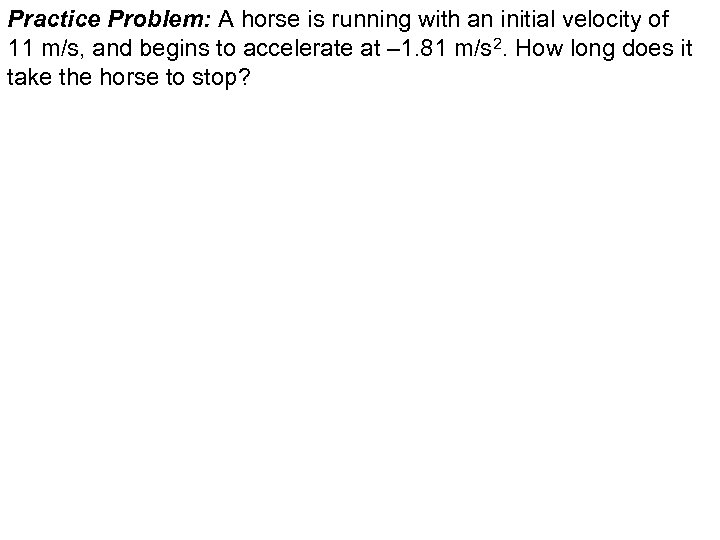Practice Problem: A horse is running with an initial velocity of 11 m/s, and begins to accelerate at – 1. 81 m/s 2. How long does it take the horse to stop?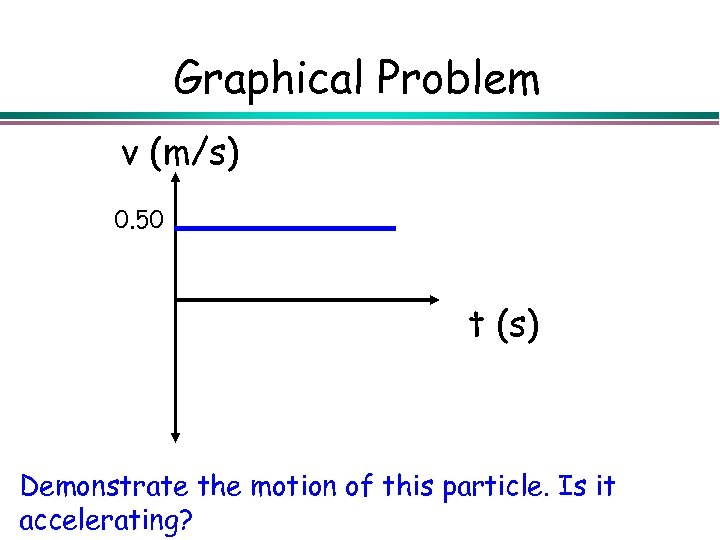Graphical Problem v (m/s) 0. 50 t (s) Demonstrate the motion of this particle. Is it accelerating?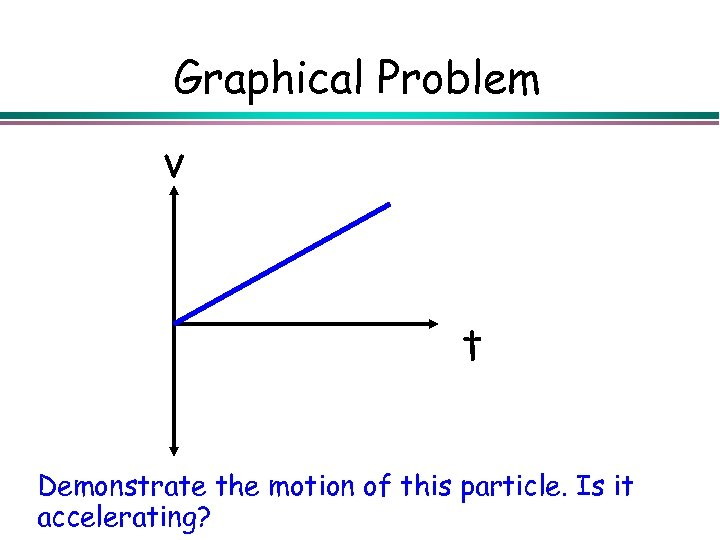Graphical Problem v t Demonstrate the motion of this particle. Is it accelerating?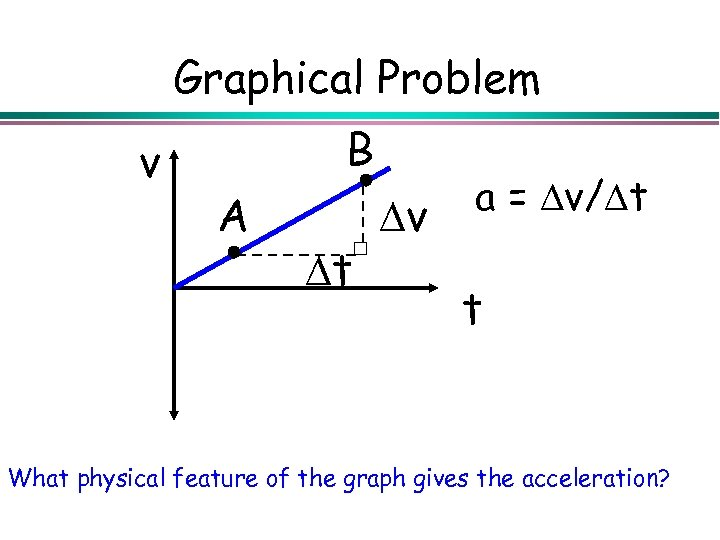Graphical Problem v B A v t a = v/ t t What physical feature of the graph gives the acceleration?Graphical Problem v B A v t a = v/ t t What physical feature of the graph gives the acceleration?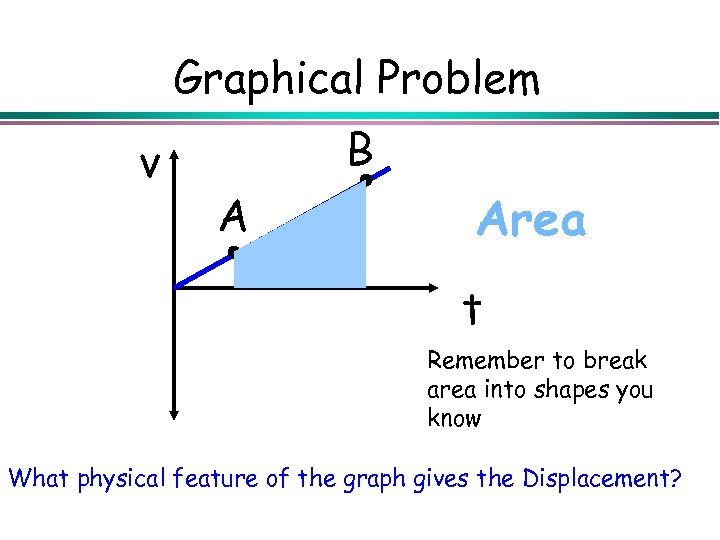Graphical Problem v B A Area t Remember to break area into shapes you know What physical feature of the graph gives the Displacement?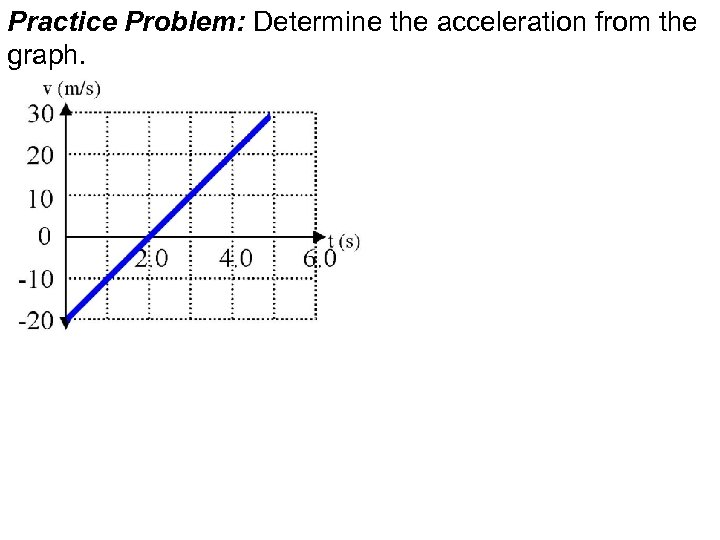Practice Problem: Determine the acceleration from the graph.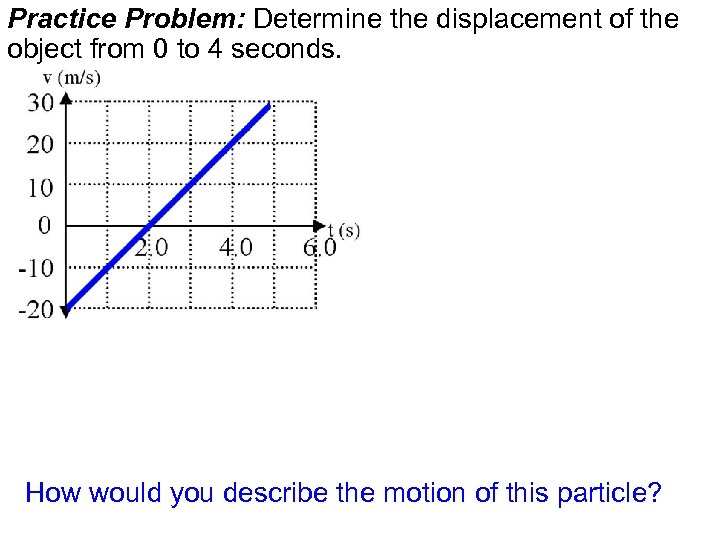Practice Problem: Determine the displacement of the object from 0 to 4 seconds. How would you describe the motion of this particle?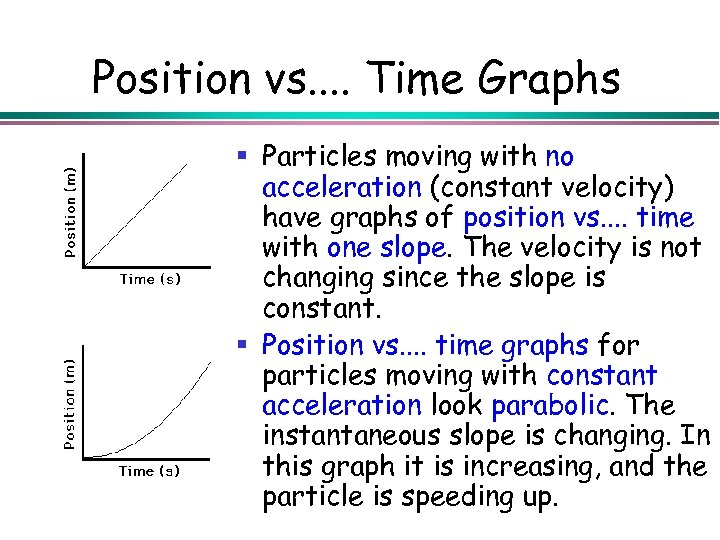Position vs. . Time Graphs § Particles moving with no acceleration (constant velocity) have graphs of position vs. . time with one slope. The velocity is not changing since the slope is constant. § Position vs. . time graphs for particles moving with constant acceleration look parabolic. The instantaneous slope is changing. In this graph it is increasing, and the particle is speeding up.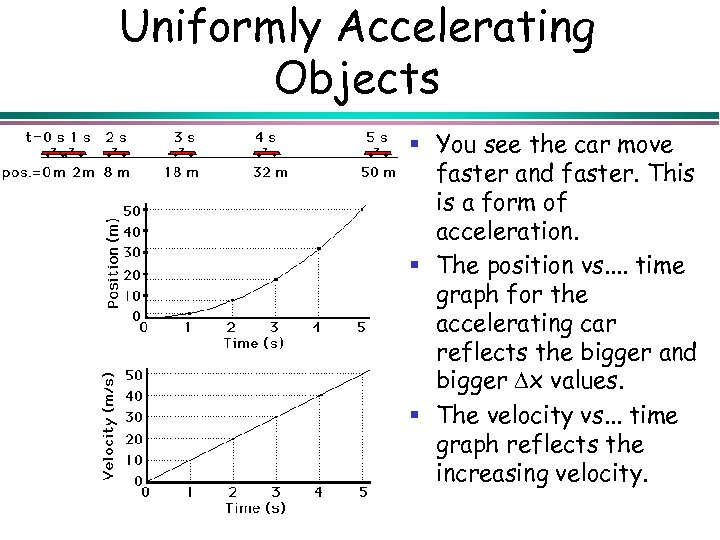Uniformly Accelerating Objects § You see the car move faster and faster. This is a form of acceleration. § The position vs. . time graph for the accelerating car reflects the bigger and bigger x values. § The velocity vs. . . time graph reflects the increasing velocity.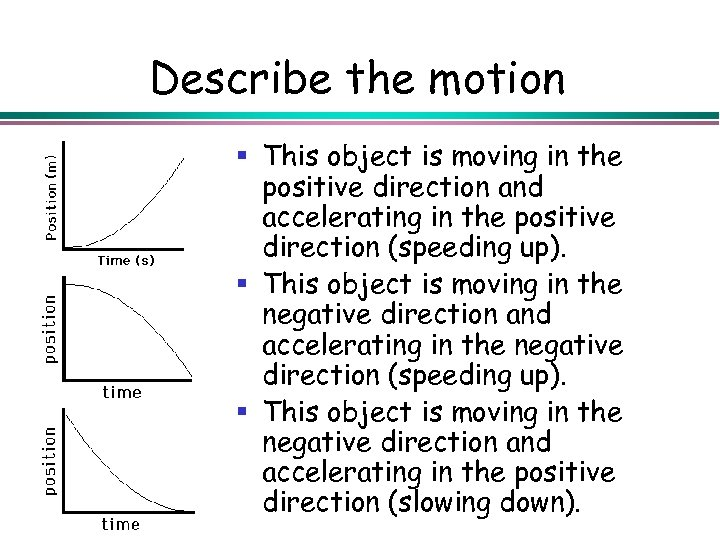Describe the motion § This object is moving in the positive direction and accelerating in the positive direction (speeding up). § This object is moving in the negative direction and accelerating in the negative direction (speeding up). § This object is moving in the negative direction and accelerating in the positive direction (slowing down).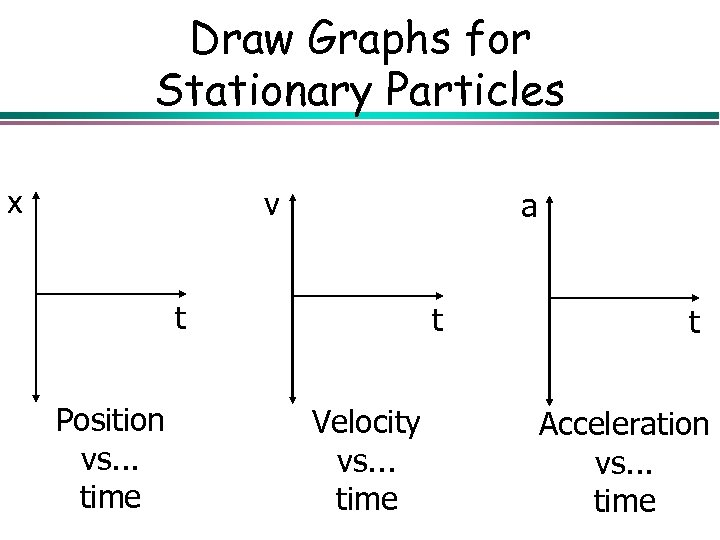Draw Graphs for Stationary Particles x v a t Position vs. . . time t Velocity vs. . . time t Acceleration vs. . . time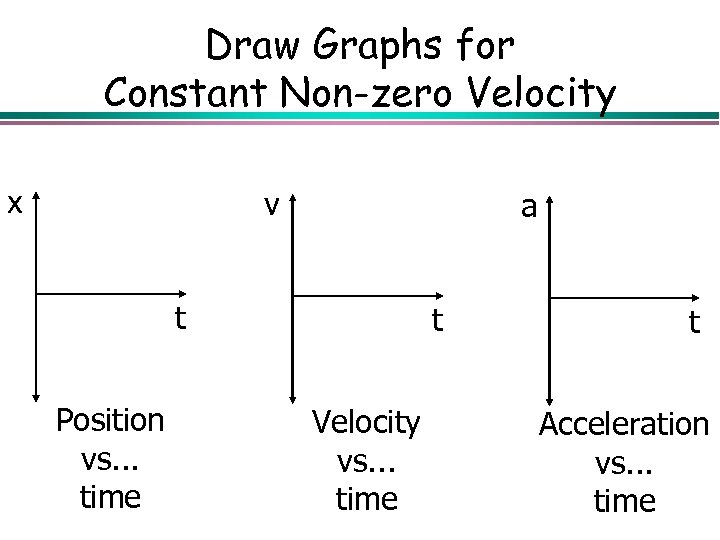Draw Graphs for Constant Non-zero Velocity x v a t Position vs. . . time t Velocity vs. . . time t Acceleration vs. . . time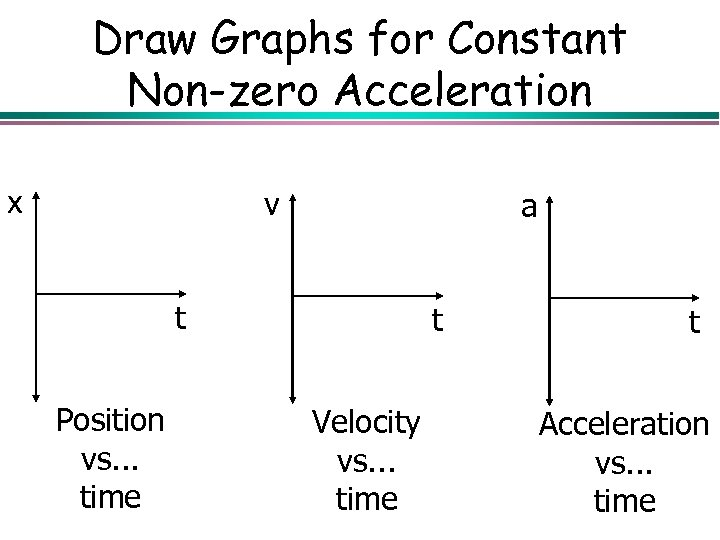Draw Graphs for Constant Non-zero Acceleration x v a t Position vs. . . time t Velocity vs. . . time t Acceleration vs. . . time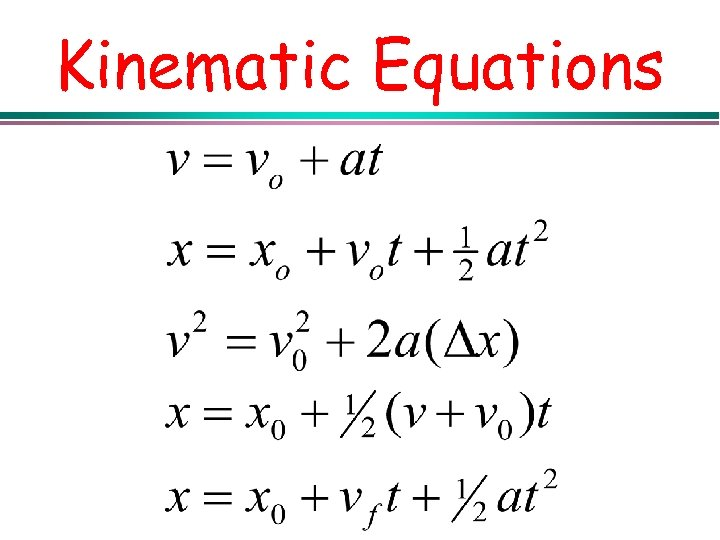Kinematic Equations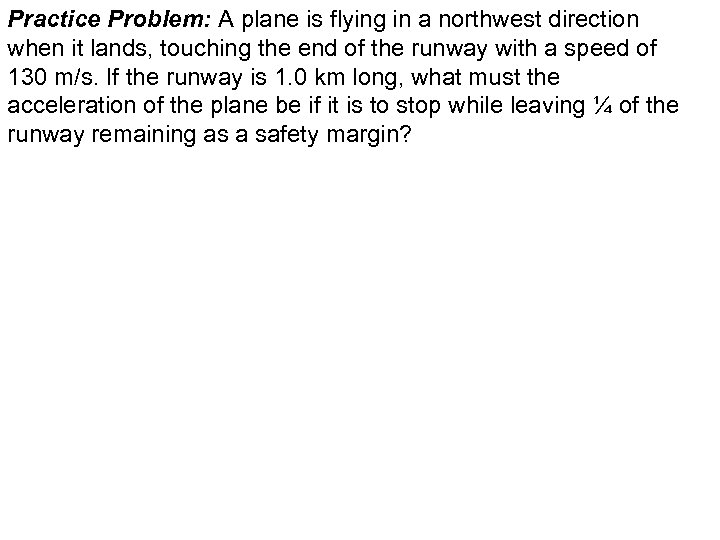Practice Problem: A plane is flying in a northwest direction when it lands, touching the end of the runway with a speed of 130 m/s. If the runway is 1. 0 km long, what must the acceleration of the plane be if it is to stop while leaving ¼ of the runway remaining as a safety margin?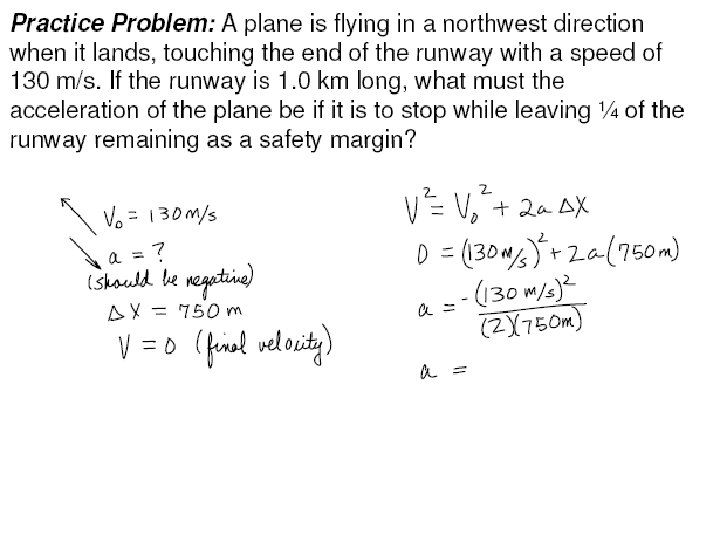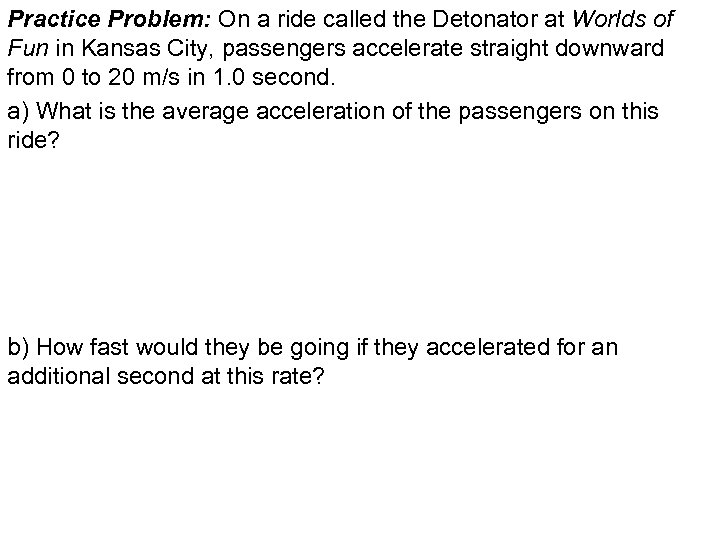Practice Problem: On a ride called the Detonator at Worlds of Fun in Kansas City, passengers accelerate straight downward from 0 to 20 m/s in 1. 0 second. a) What is the average acceleration of the passengers on this ride? b) How fast would they be going if they accelerated for an additional second at this rate?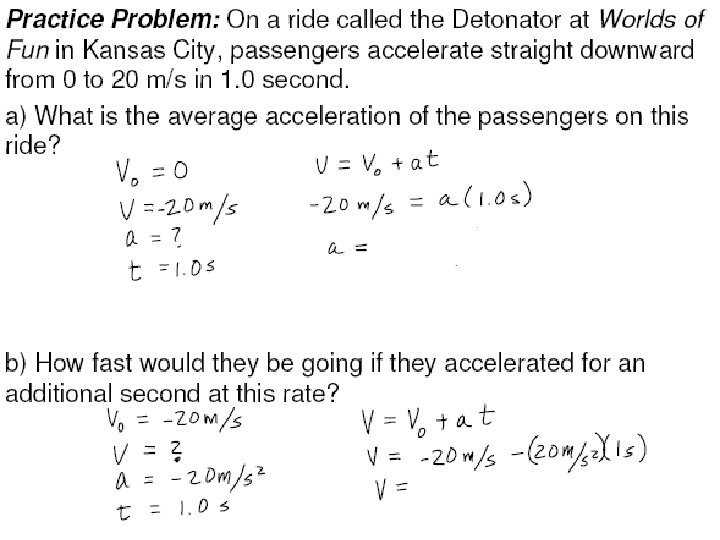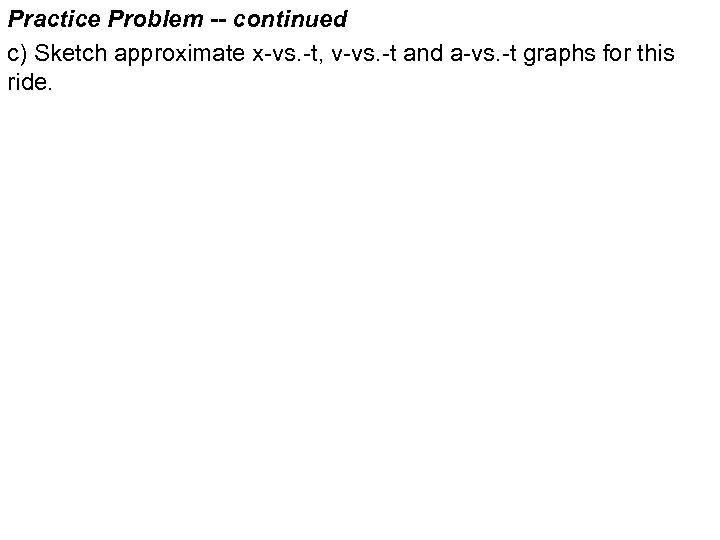Practice Problem -- continued c) Sketch approximate x-vs. -t, v-vs. -t and a-vs. -t graphs for this ride.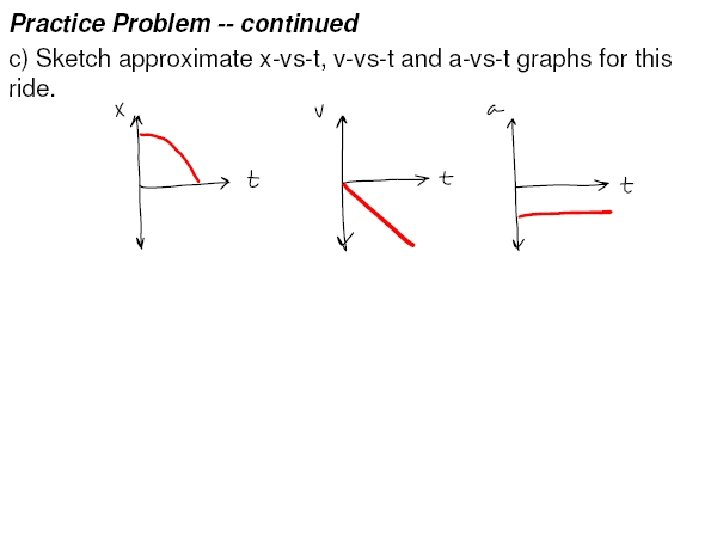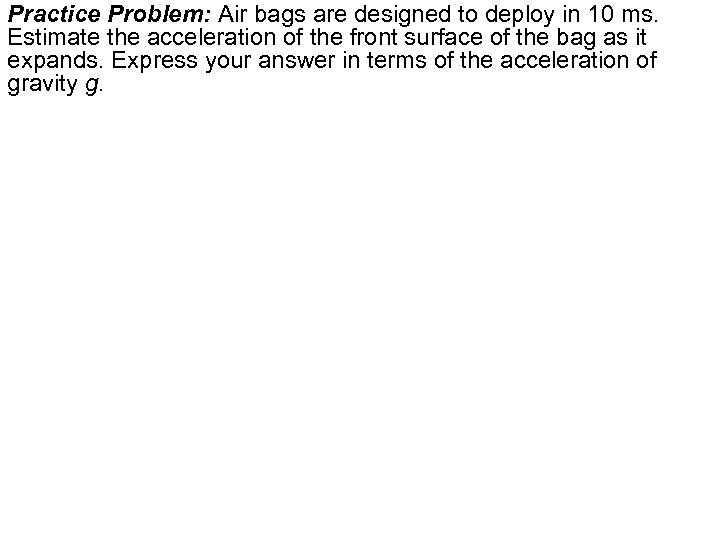Practice Problem: Air bags are designed to deploy in 10 ms. Estimate the acceleration of the front surface of the bag as it expands. Express your answer in terms of the acceleration of gravity g.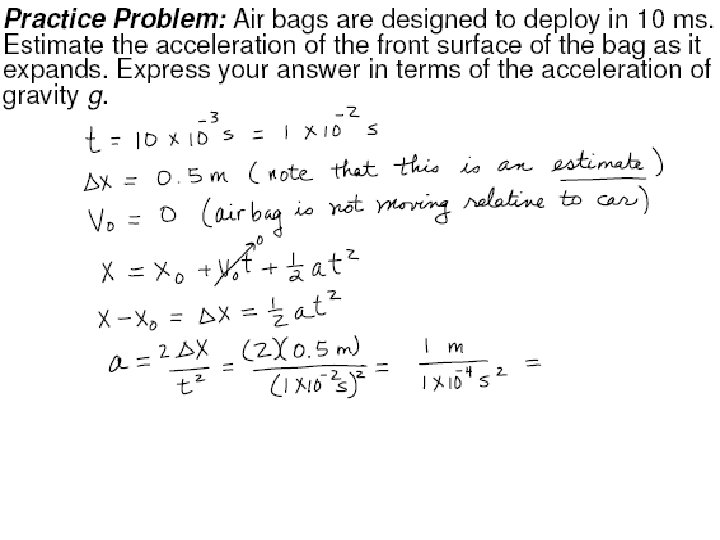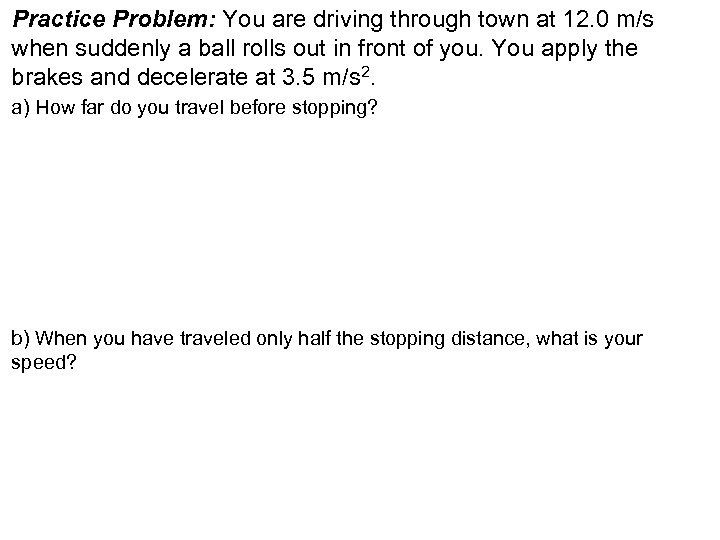Practice Problem: You are driving through town at 12. 0 m/s when suddenly a ball rolls out in front of you. You apply the brakes and decelerate at 3. 5 m/s 2. a) How far do you travel before stopping? b) When you have traveled only half the stopping distance, what is your speed?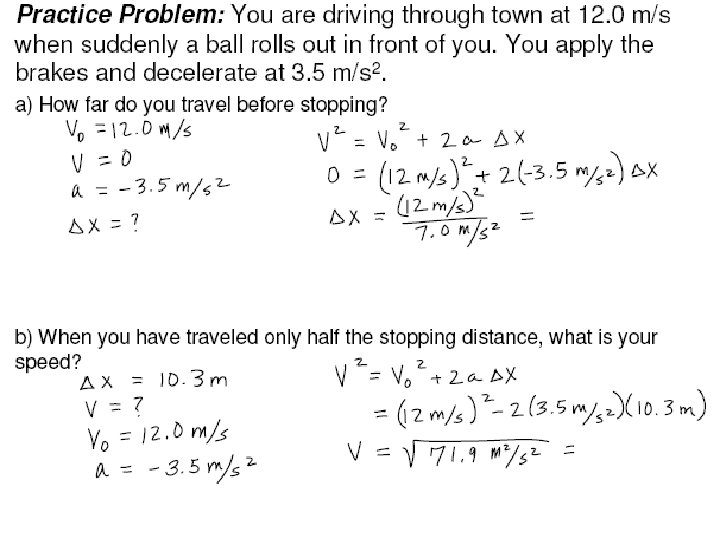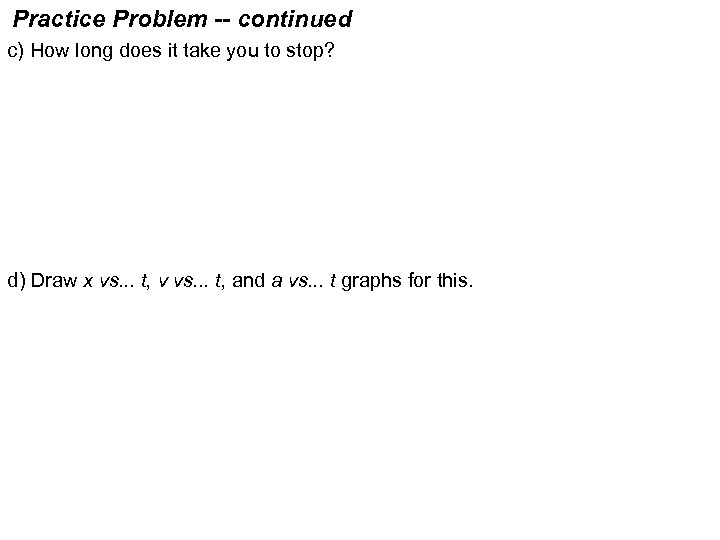Practice Problem -- continued c) How long does it take you to stop? d) Draw x vs. . . t, v vs. . . t, and a vs. . . t graphs for this.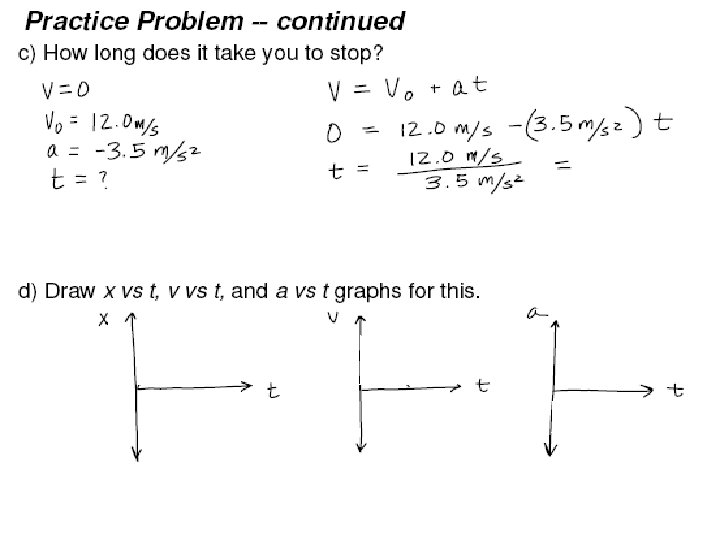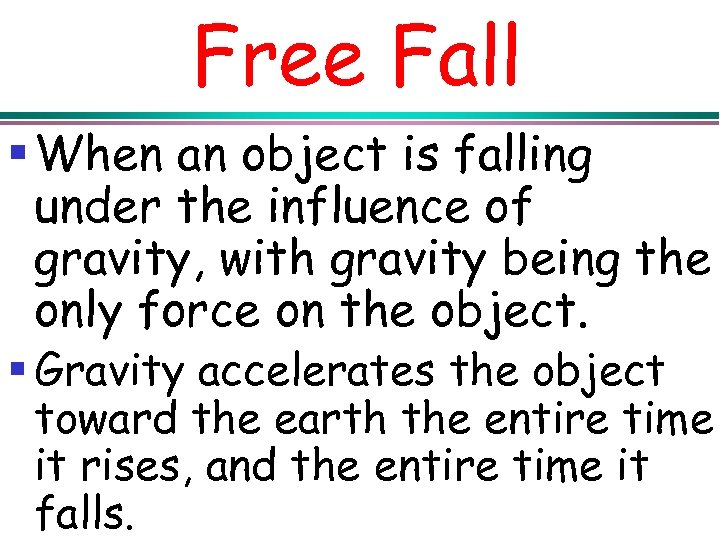Free Fall § When an object is falling under the influence of gravity, with gravity being the only force on the object. § Gravity accelerates the object toward the earth the entire time it rises, and the entire time it falls.§ The acceleration due to gravity near the surface of the earth has a magnitude of 2. The direction of 9. 81 m/s this acceleration is DOWN. § Air resistance is ignored.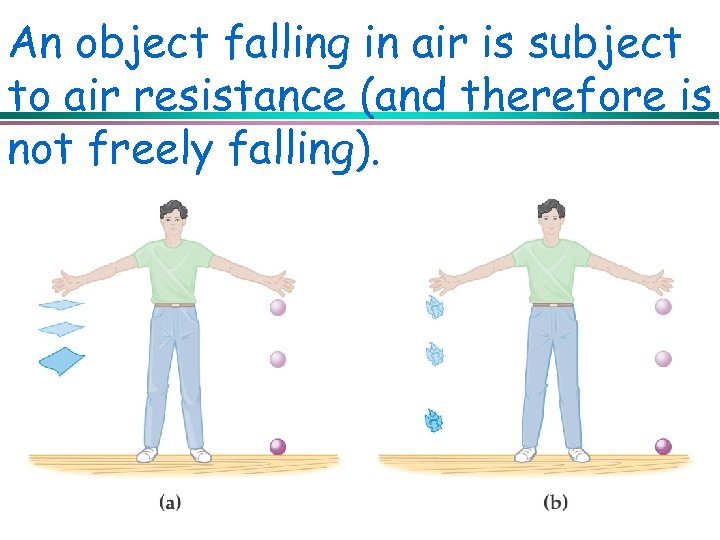An object falling in air is subject to air resistance (and therefore is not freely falling).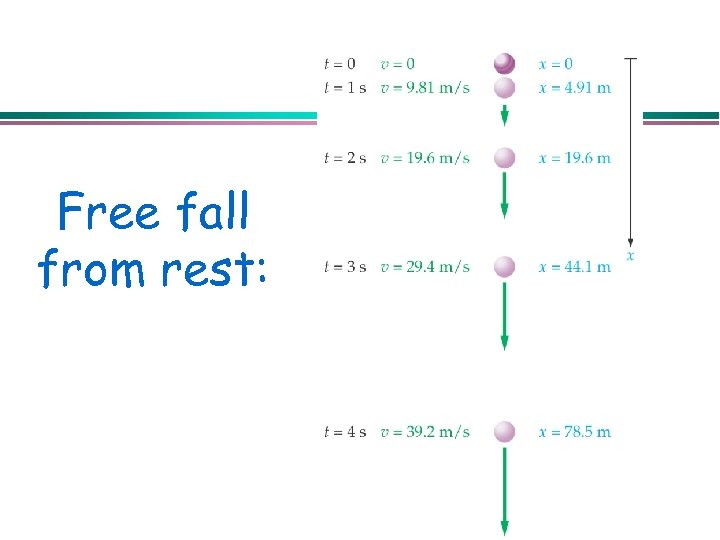Free fall from rest: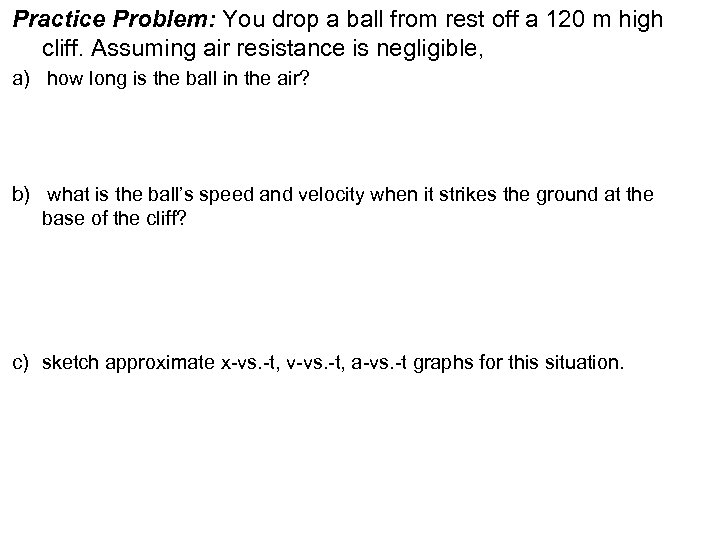Practice Problem: You drop a ball from rest off a 120 m high cliff. Assuming air resistance is negligible, a) how long is the ball in the air? b) what is the ball’s speed and velocity when it strikes the ground at the base of the cliff? c) sketch approximate x-vs. -t, v-vs. -t, a-vs. -t graphs for this situation.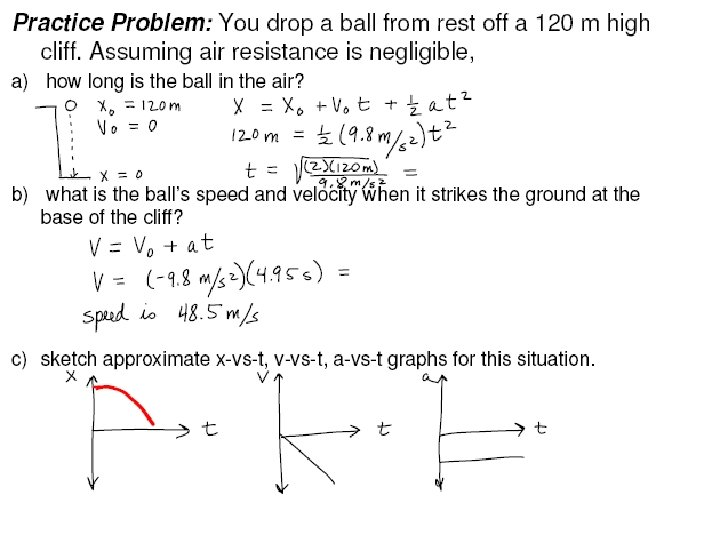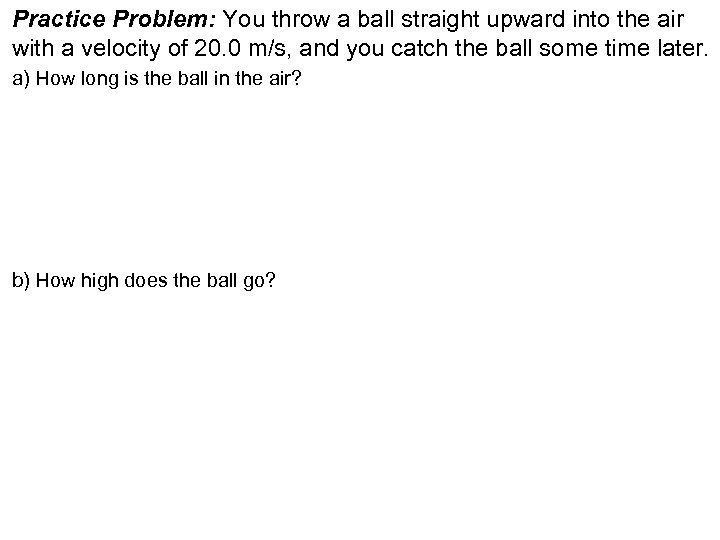Practice Problem: You throw a ball straight upward into the air with a velocity of 20. 0 m/s, and you catch the ball some time later. a) How long is the ball in the air? b) How high does the ball go?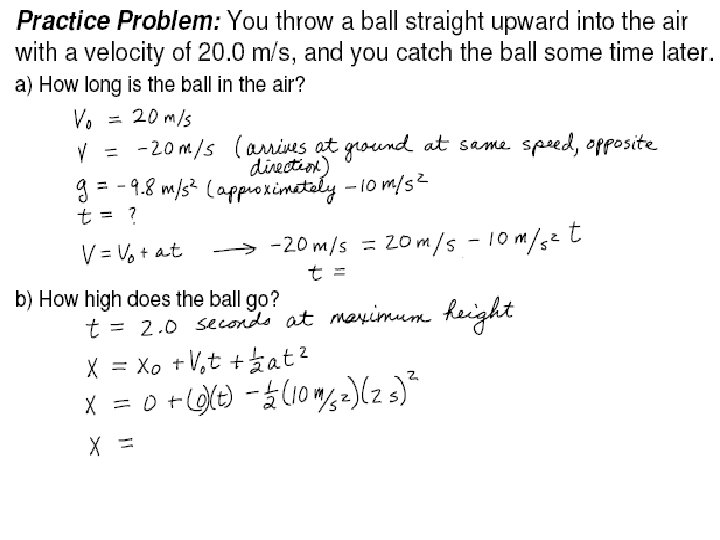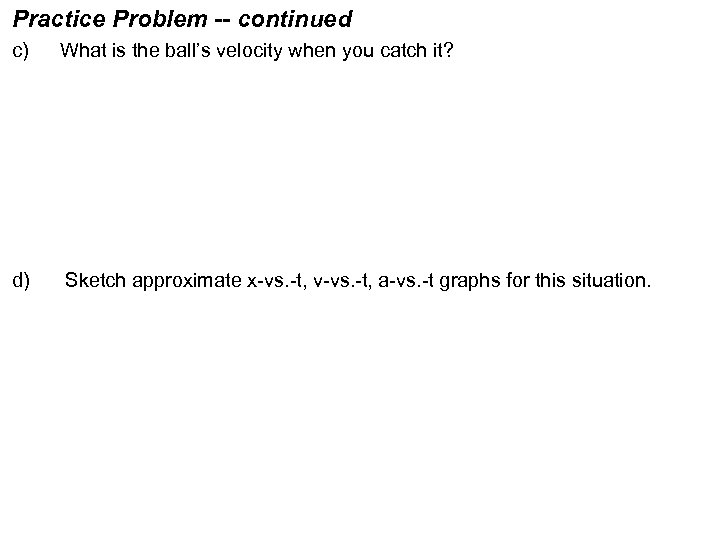Practice Problem -- continued c) What is the ball’s velocity when you catch it? d) Sketch approximate x-vs. -t, v-vs. -t, a-vs. -t graphs for this situation.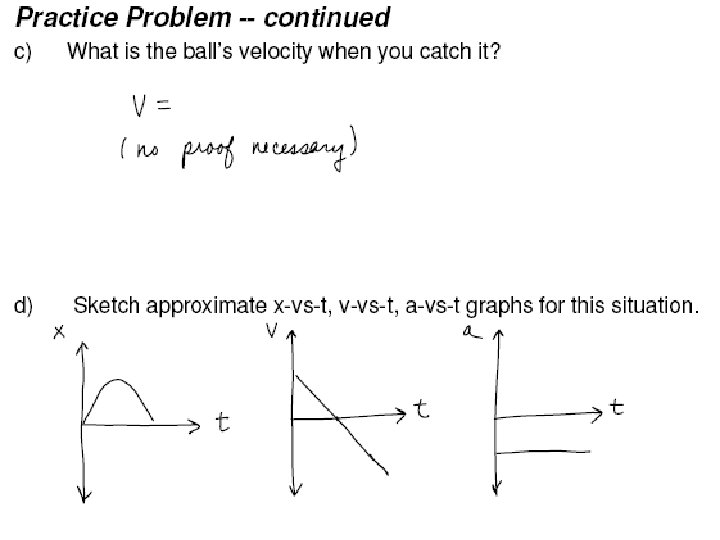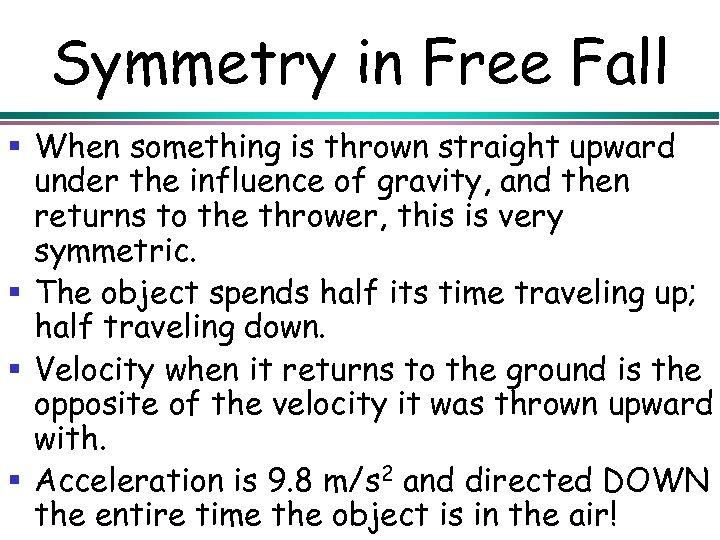Symmetry in Free Fall § When something is thrown straight upward under the influence of gravity, and then returns to the thrower, this is very symmetric. § The object spends half its time traveling up; half traveling down. § Velocity when it returns to the ground is the opposite of the velocity it was thrown upward with. § Acceleration is 9. 8 m/s 2 and directed DOWN the entire time the object is in the air!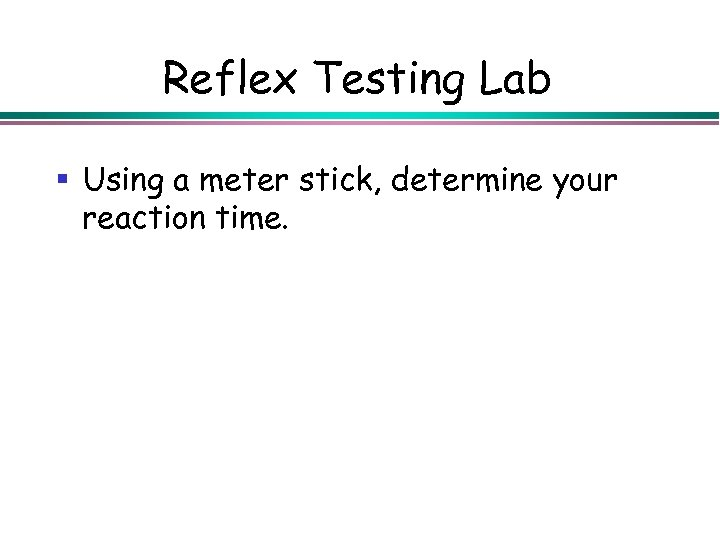Reflex Testing Lab § Using a meter stick, determine your reaction time.